# Probability Density Functions, Part 2

In a previous posting, I showed some plots that displayed the probability density functions (or PDF) of a number of commercial audio recordings. (If you are new to the concept of a probability density function, then you might want to at least have a look at that posting before reading further…)

I’ve been doing a little more work on this subject, with some possible implications on how to interpret those plots. Or, perhaps more specifically, with some possible implications on possible conclusions to be drawn from those plots.

# Full-band examples

To start, let’s create some noise with a desired PDF, without imposing any frequency limitations on the signal.

To do this, I’ve ported equations from “Computer Music: Synthesis, Composition, and Performance” by Charles Dodge and Thomas A. Jerse, Schirmer Books, New York (1985) to Matlab. That code is shown below in italics, in case you might want to use it. (No promises are made regarding the code quality… However, I will say that I’ve written the code to be easily understandable, rather than efficient – so don’t make fun of me.) I’ve made the length of the noise samples 2^16 because I like that number. (Actually, it’s for other reasons involving plotting the results of an FFT, and my own laziness regarding frequency scaling – but that’s my business.)

## Uniform (aka Rectangular) Distribution

uniform = rand(2^16, 1);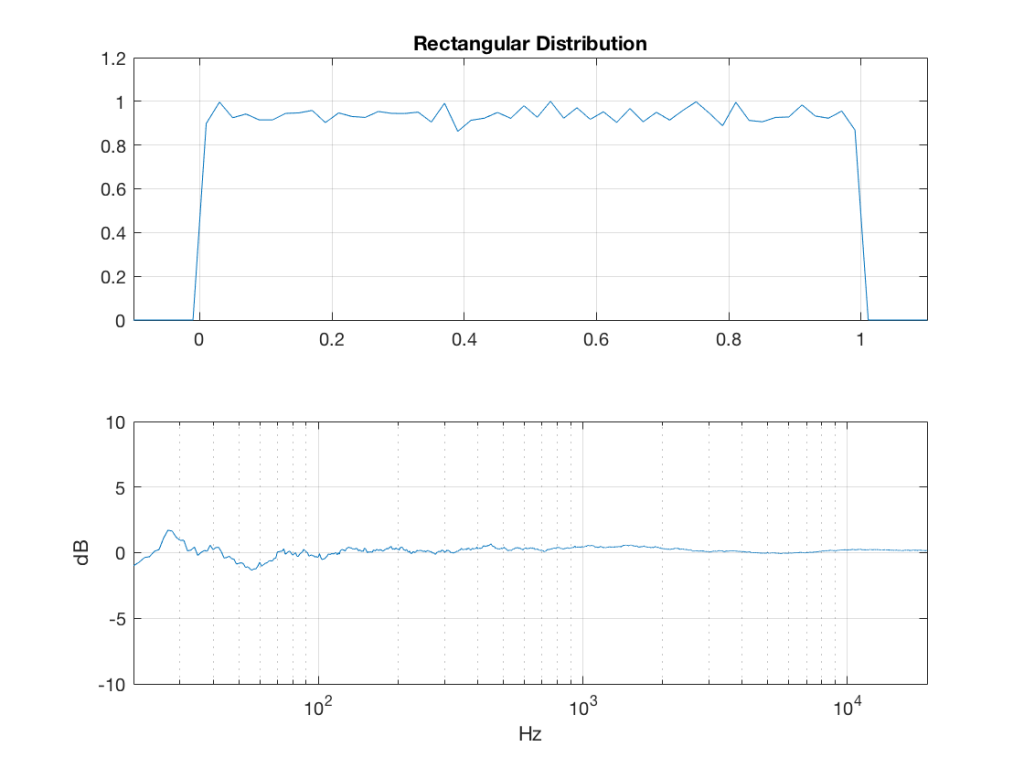Fig 1: The PDF and the spectrum (1-octave smoothed) of a noise signal with a rectangular distribution. Note that there is a DC component, since there are no negative values in the signal.

Of course, as you can see in the plots in Figure 1, the signal is not “perfectly” rectangular, nor is it “perfectly” flat. This is because it’s noise. If I ran exactly the same code again, the result would be different, but also neither perfectly rectangular nor flat. Of course, if I ran the code repeatedly, and averaged the results,  the average would become “better” and “better”.

## Linear Distribution

linear_temp_1 = rand(2^16, 1);

linear_temp_2  = rand(2^16, 1);

temp_indices = find(linear_temp_1 < linear_temp_2);

linear = linear_temp_2;

linear(temp_indices) = linear_temp_1(temp_indices);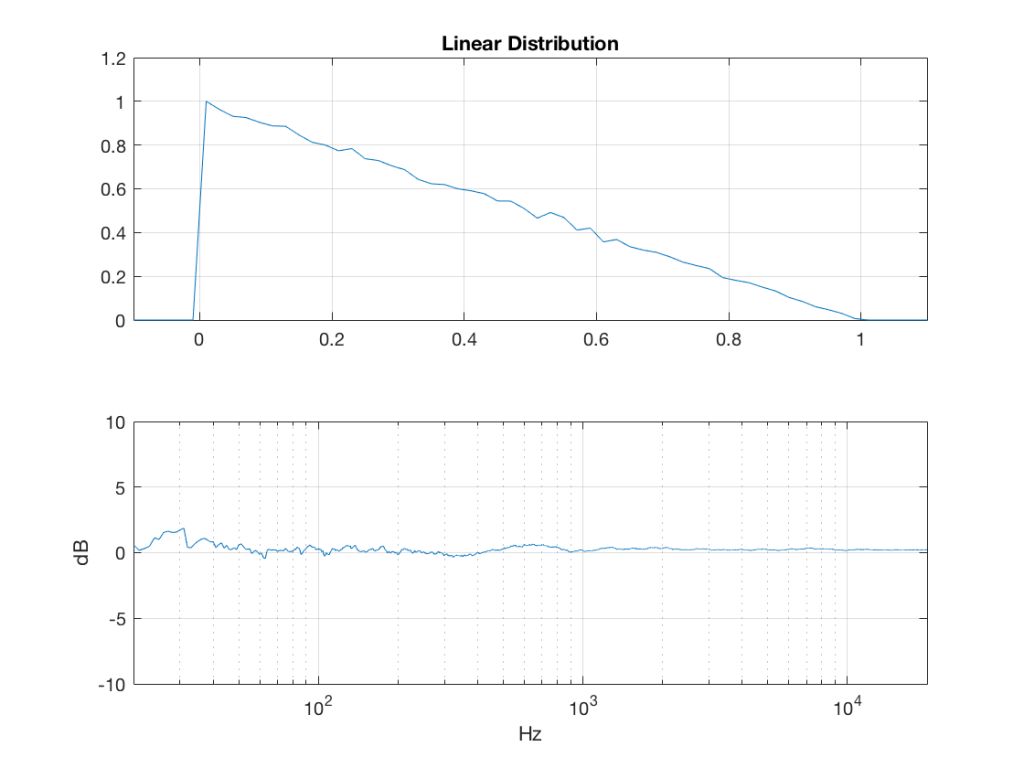Fig 2: The PDF and the spectrum (1-octave smoothed) of a noise signal with a linear distribution. Note that there is a DC component, since there are no negative values in the signal.

## Triangular Distribution

triangular = rand(2^16, 1) – rand(2^16, 1);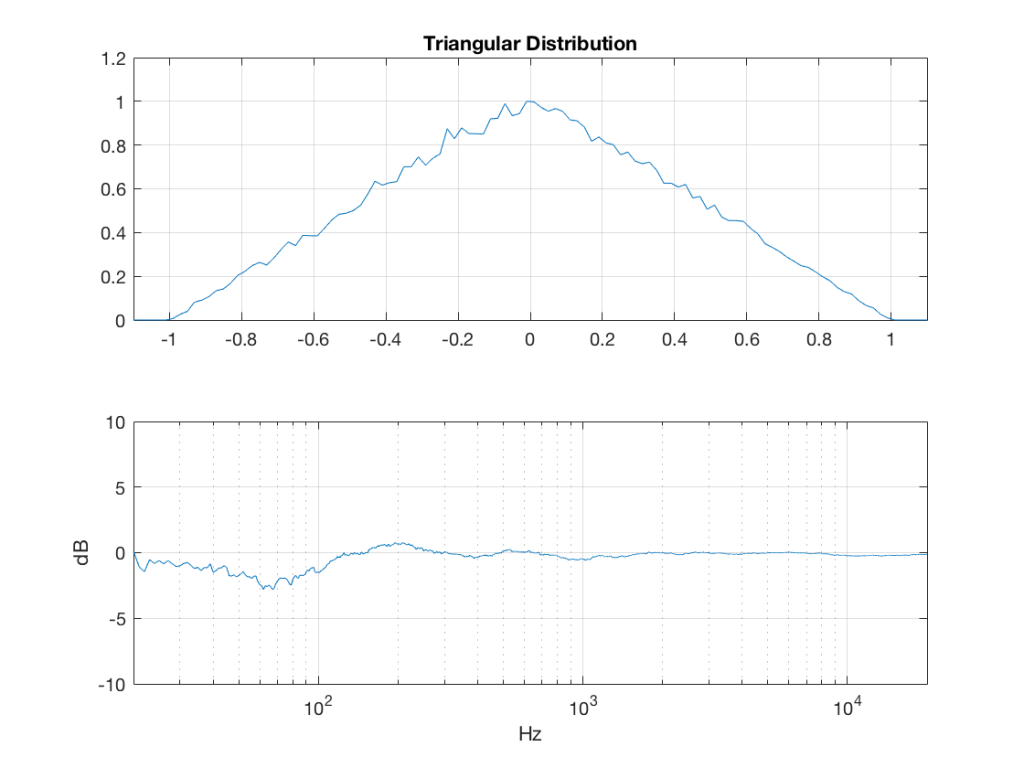Fig 3: The PDF and the spectrum (1-octave smoothed) of a rise signal with a triangular distribution. Note that there is no DC component, since the PDF is symmetrical across the 0 line.

## Exponential Distribution

lambda = 1;     % lambda must be greater than 0

exponential_temp = rand(2^16, 1) / lambda;

if any(exponential_temp == 0)    % ensure that no values of exponential_temp are 0

end

exponential = -log(exponential_temp);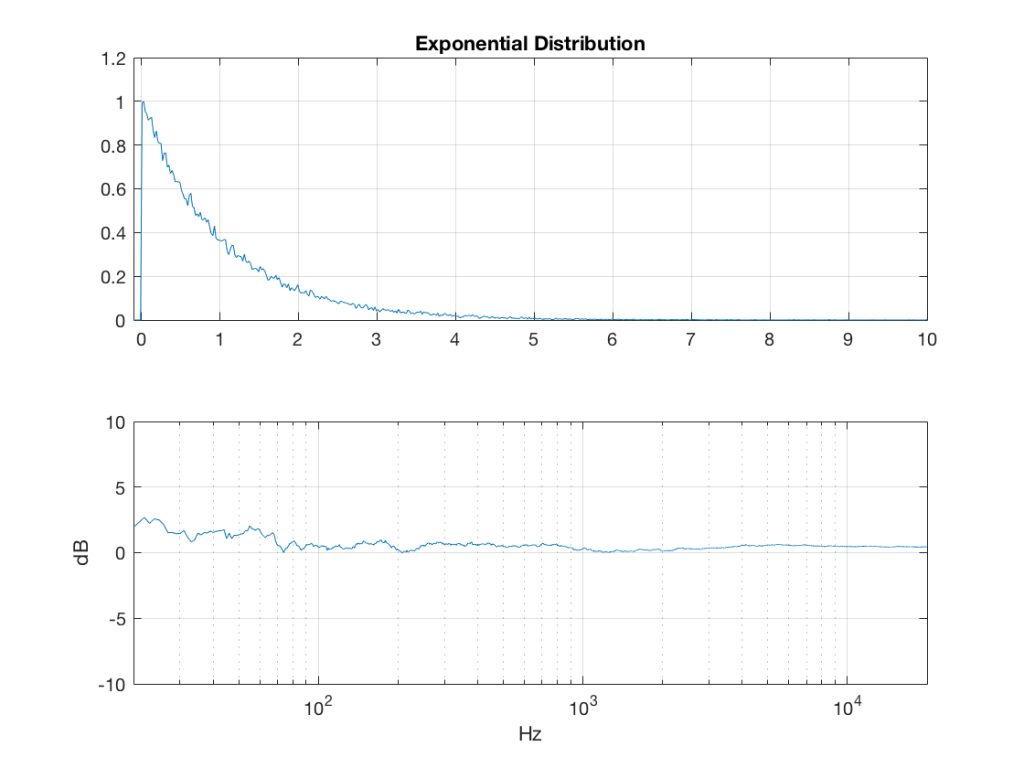Fig 4: The PDF and the spectrum (1-octave smoothed) of a rise signal with an exponential distribution. Note that there is a DC component, since there are no negative values in the signal. Note as well that the values can be significantly higher than 1, so you might incur clipping if you use this without thinking…

## Bilateral Exponential Distribution (aka Laplacian)

lambda = 1;     % must be greater than 0

bilex_temp = 2 * rand(2^16, 1);

% check that no values of bilex_temp are 0 or 2

if any(bilex_temp == 0)

end

bilex_lessthan1 = find(bilex_temp <= 1);

bilex(bilex_lessthan1, 1) = log(bilex_temp(bilex_lessthan1)) / lambda;

bilex_greaterthan1 = find(bilex_temp > 1);

bilex_temp(bilex_greaterthan1) = 2 – bilex_temp(bilex_greaterthan1);

bilex(bilex_greaterthan1, 1) = -log(bilex_temp(bilex_greaterthan1)) / lambda;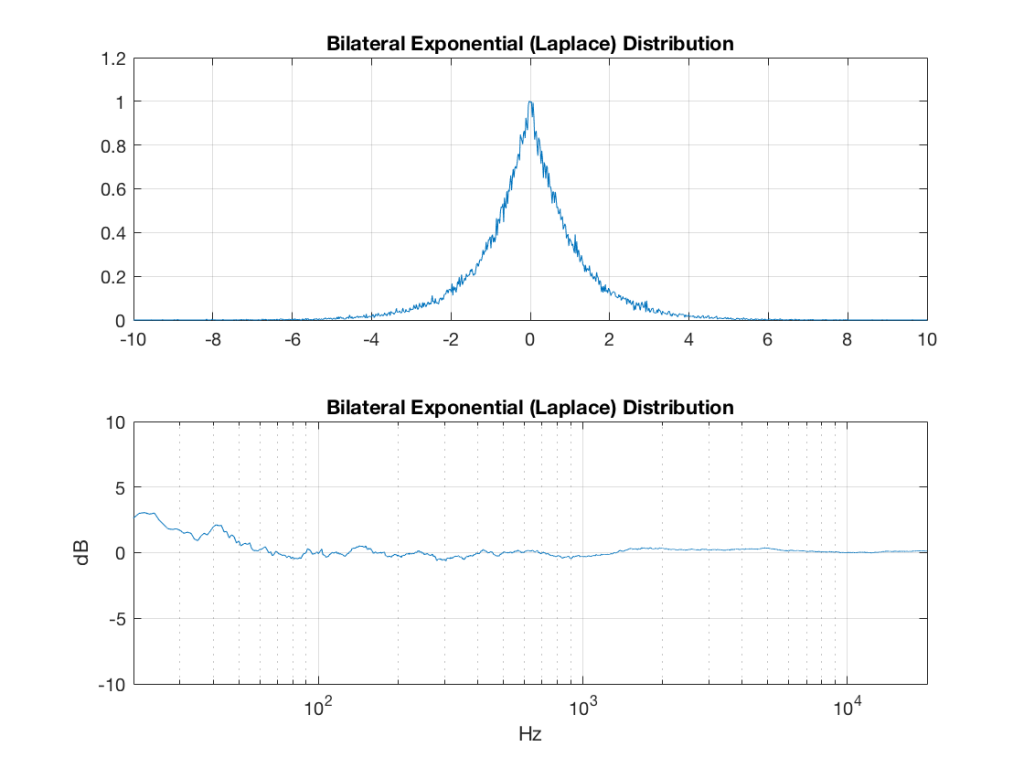Fig 5: The PDF and the spectrum (1-octave smoothed) of a rise signal with a bilateral exponential distribution. Note that there is no DC component, since the PDF is symmetrical across the 0 line. Note as well that the values can be significantly higher than 1 (or less than -1), so you might incur clipping if you use this without thinking…

## Gaussian

sigma = 1;

xmu = 0;   % offset

n = 100; % number of random number vectors used to create final vector (more is better)

xnover = n/2;

sc = 1/sqrt(n/12);

total = sum(rand(2^16, n), 2);

gaussian = sigma * sc * (total – xnover) + xmu;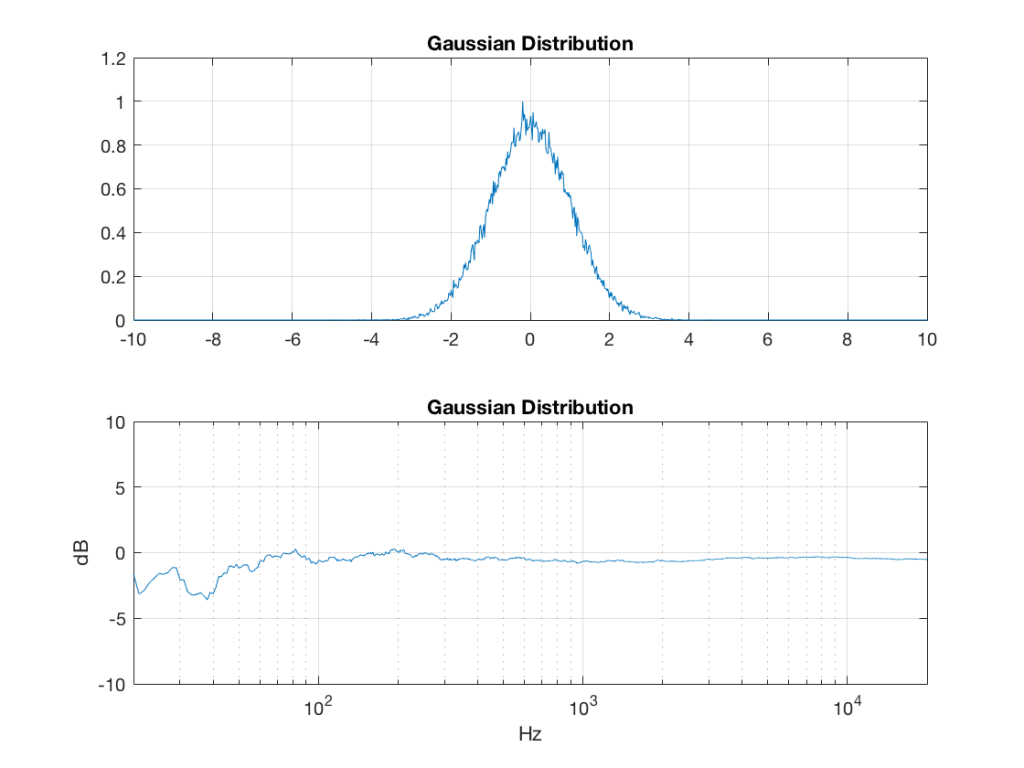Fig 6:  The PDF and the spectrum (1-octave smoothed) of a rise signal with a Gaussian distribution. Note that there is no DC component, since the PDF is symmetrical across the 0 line. Note as well that the values can be significantly higher than 1 (or less than -1), so you might incur clipping if you use this without thinking…

Of course, if you are using Matlab, there is an easier way to get a noise signal with a Gaussian PDF, and that is to use the randn() function.

# The effects of band-passing the signals

What happens to the probability distribution of the signals if we band-limit them? For example, let’s take the signals that were plotted above, and put them through two sets of two second-order Butterworth filters in series, one set producing a high-pass filter at 200 Hz and the other resulting in a low-pass filter at 2 kHz .(This is the same as if we were making a mid-range signal in a 4th-order Linkwitz-Riley crossover, assuming that our midrange drivers had flat magnitude responses far beyond our crossover frequencies, and therefore required no correction in the crossover…)

What happens to our PDF’s as a result of the band limiting? Let’s see…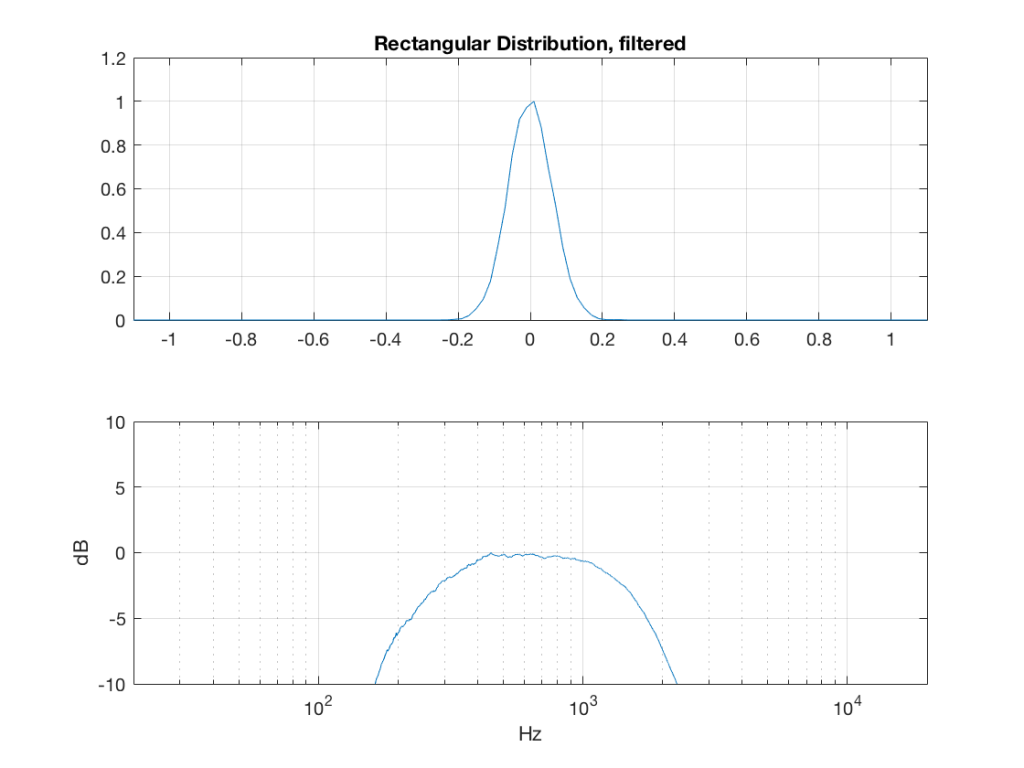Fig 7: The PDF of noise with a rectangular distribution that has been band-limited from 200 Hz to 2 kHz.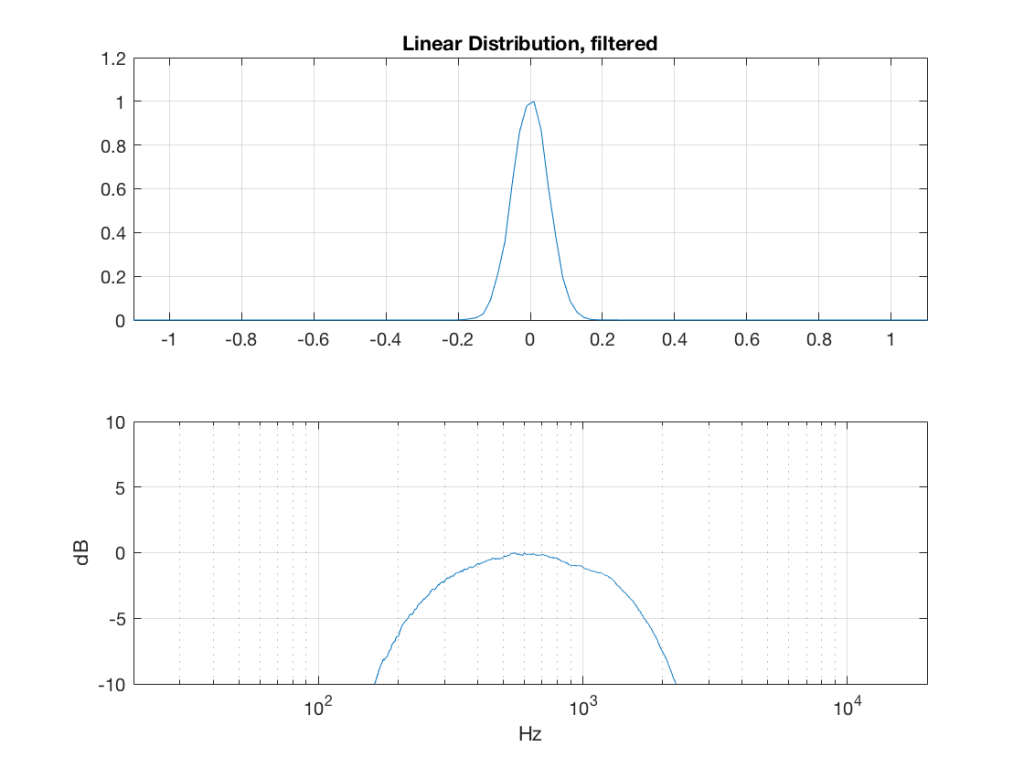Fig 8: The PDF of noise with a linear distribution that has been band-limited from 200 Hz to 2 kHz.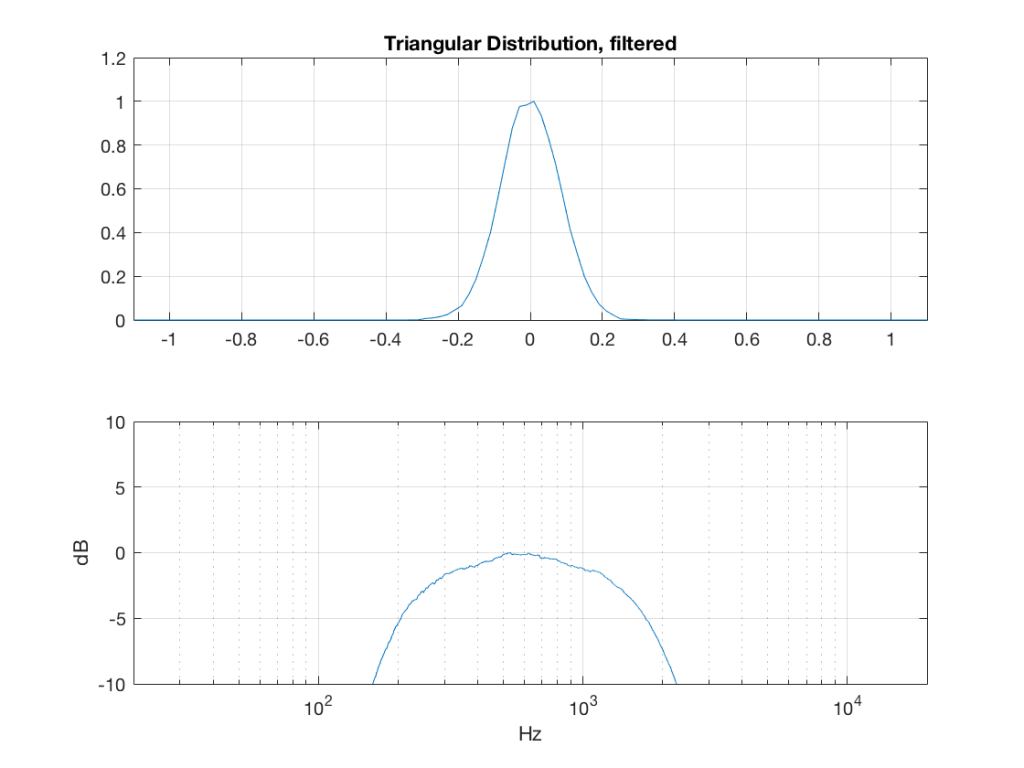Fig 9: The PDF of noise with a triangular distribution that has been band-limited from 200 Hz to 2 kHz.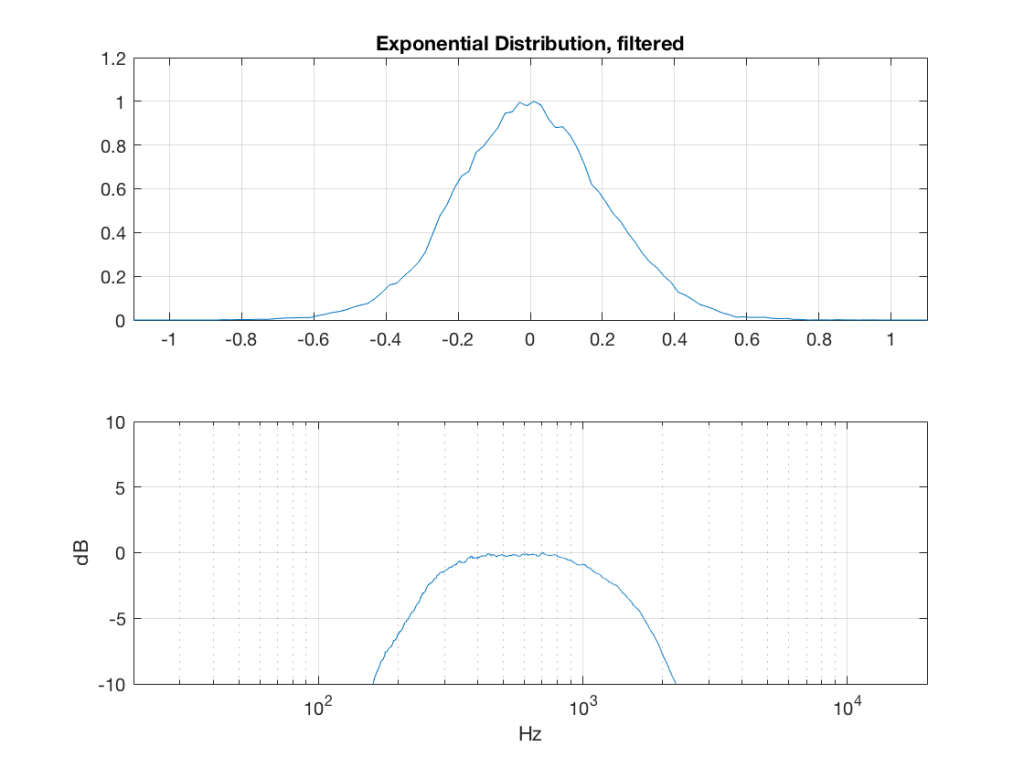Fig 10: The PDF of noise with an exponential distribution that has been band-limited from 200 Hz to 2 kHz.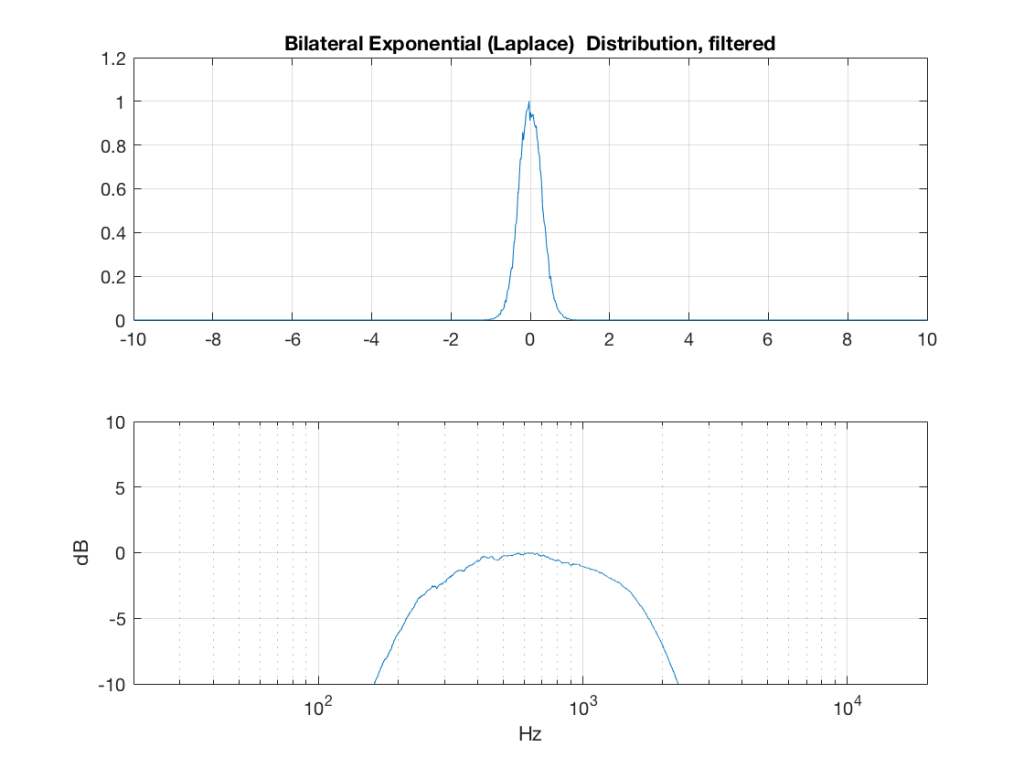Fig 11: The PDF of noise with a Laplacian distribution that has been band-limited from 200 Hz to 2 kHz.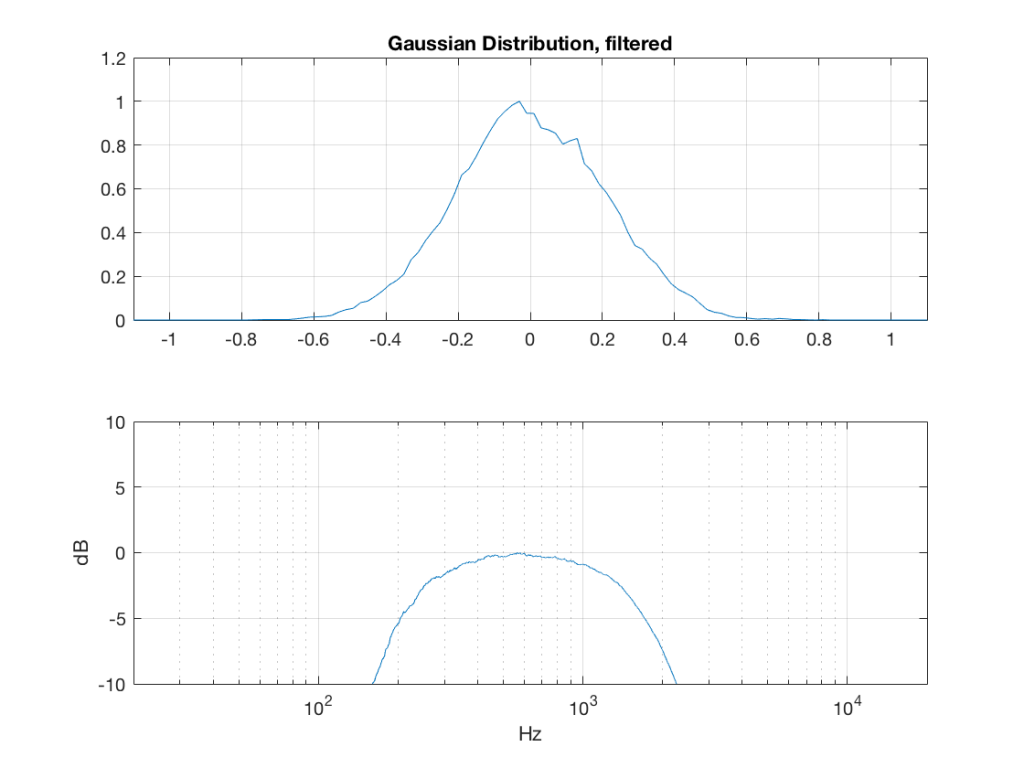Fig 12: The PDF of noise with a Gaussian distribution that has been band-limited from 200 Hz to 2 kHz.

So, what we can see in Figures 7 through 12 (inclusive) is that, regardless of the original PDF of the signal, if you band-limit it, the result has a Gaussian distribution.

And yes, I tried other bandwidths and filter slopes. The result, generally speaking, is the same.

One part of this effect is a little obvious. The high-pass filter (in this case, at 200 Hz) removes the DC component, which makes all of the PDF’s symmetrical around the 0 line.

However, the “punch line” is that, regardless of the distribution of the signal coming into your system (and that can be quite different from song to song as I showed in this posting) the PDF of the signal after band-limiting (say, being sent to your loudspeaker drivers) will be Gaussian-ish.

And, before you ask, “what if you had only put in a high-pass or a low-pass filter?” – that answer is coming in a later posting…

## Post-script

This posting has a Part 1 that you’ll find here, and a Part 3 that you’ll find here.

# B&O Tech: BeoLab 90 – How to set up Active Room Calibration

#64 in a series of articles about the technology behind Bang & Olufsen products

# Clinging to long-standing fallacies

Stephen Colbert once said that George W. Bush was a man of conviction. He believed the same thing on Wednesday that he believed on Monday, no matter what happened on Tuesday….

Often people ask me questions about sound quality. In “the old days”, it was something like “which is better, analogue or digital?” Later, it became “which is better, MP3 or Ogg Vorbis (or something else)?”. These days, it’s something like “which streaming service has the best quality?” or “Is high-resolution audio really worth it?” Or, it’s a more general question like “what loudspeaker (or headphones) would you recommend?”

My answer to these questions is always the same. It’s a combination of “it depends….” and “whatever I tell you today, it might be different tomorrow…”Something that is true on Monday may not still be true on Wednesday…

Recently, during a discussion about something else, I told someone that many, if not most, mobile devices will clip (and therefore) distort a signal if you try to boost it (e.g. with a “bass boost” or a “pop” setting instead of playing the signals “flat”), but they won’t if you cut. Therefore, on a mobile device, it’s smarter to cut than to boost a signal.

I made that statement based on some past measurements that were done that showed that, if you put a 0 dB FS signal at a low frequency (say, 80 Hz) on different mobile devices, and turned the “bass boost” (or equivalent, such as a “pop” or “rock” setting) on, the signal would be often be clipped, and therefore it would increase the level of distortion – sometimes quite dramatically. The measurements also showed that this was independent of volume setting. So, turning down the level didn’t help – it just made things quieter, but maintained the same THD value. This is likely because the processing in such devices & software was done (a) before the volume control and (b) in a fixed-point system that does not have a carefully-managed headroom.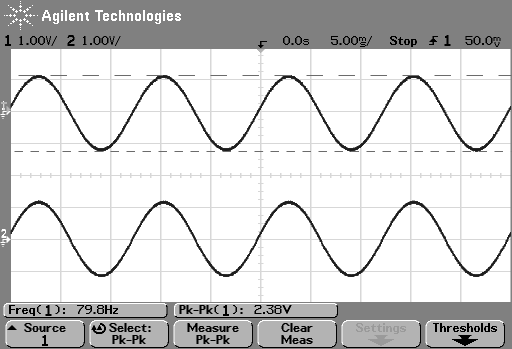Fig 1. The minijack headphone output of a mobile device that was measured a long time (about 2 years) ago. This was done at a maximum volume setting  (notice the reading of 2.38 V peak-to-peak in the centre bottom of the display) playing a .wav file with an 80 Hz sine wave (displayed on the bottom left). The EQ in the device was set to “Flat”.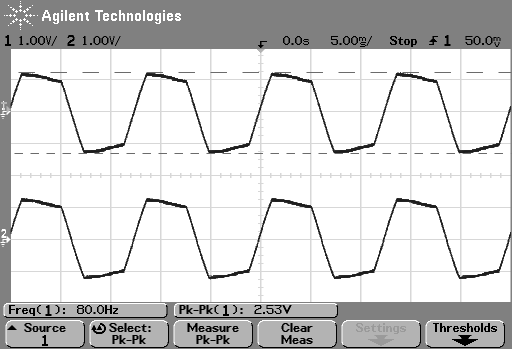Fig 2. The output of the same device at the same maximum volume setting, The EQ was set to boost the bass signals. Notice that the signal is now clipped.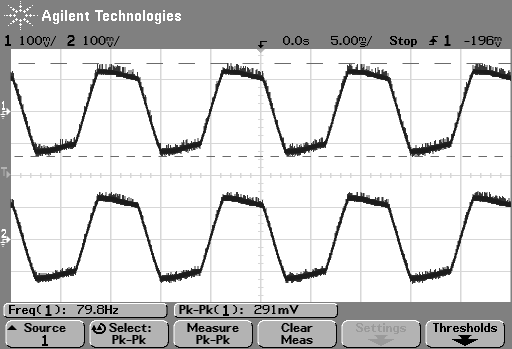Fig 3. The output of the same device at a lower volume setting (notice that the Peak-to-Peak measurement shows 291 mV on the centre bottom of the display), The EQ was set to boost the bass signals. Notice that the signal is still clipped as much as it was at a high volume setting – only a little extra noise is visible, because we’re closer to the noise floor of the device.

So, just to be sure that this was still true on newer devices and software, I did a couple of quick measurements. I put a logartihmically-swept sine wave with a level of 0 dB FS and a frequency range of 20 Hz to 2 kHz on a current mobile audio device with a minijack headphone output. With the output volume at maximum and the EQ set to “OFF” or “FLAT”, I recorded the output and did a little analysis.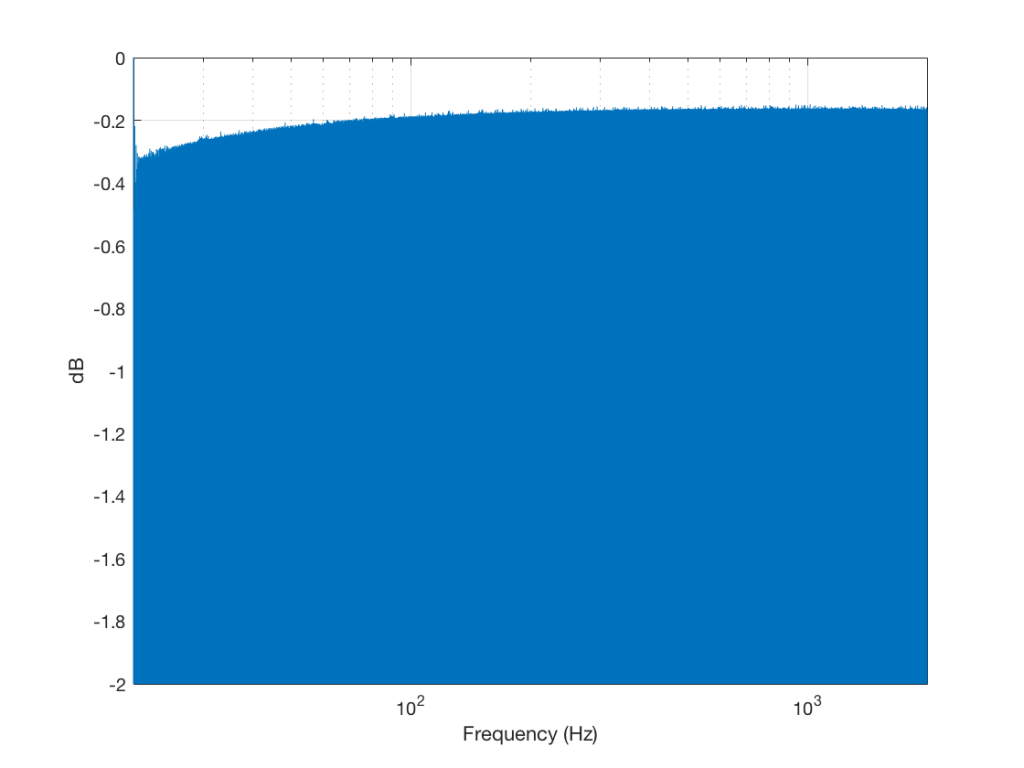Fig 4. A plot of the absolute value of the signal as it is swept in frequency from 20 Hz to 2000 Hz. Notice that the y-axis is zoomed in to a total range of only 2 dB.

Although Figure 4 is a plot of the time-domain output of the system (in other words, I’m just plotting 20*log10(abs(signal)), it can be read as a frequency response plot (which is why I’ve labelled it that way on the x-axis). Actually, though, I have to be explicit and say that we’re actually looking at the absolute value of the signal itself, and the y-axis is labelled as the frequency at the time of the signal coming in.

If we zoom in on the signal, we get the plot shown in Figure 5.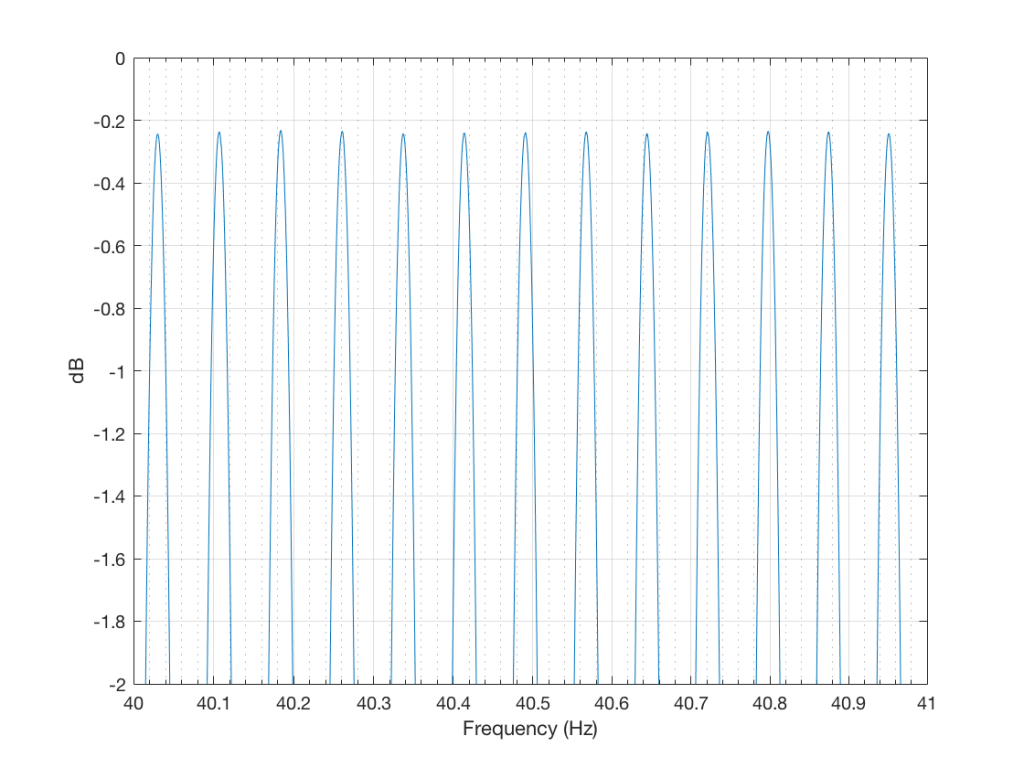Fig 5. Zooming in to the plot in figure 1, when the signal is sweeping between 40 Hz and 41 Hz. I’m looking for clipping, but I don’t see anything worth worrying about.

Then I turned the bass boost setting to “ON” and repeated the recording, without changing anything else. The result is shown in Figure 6.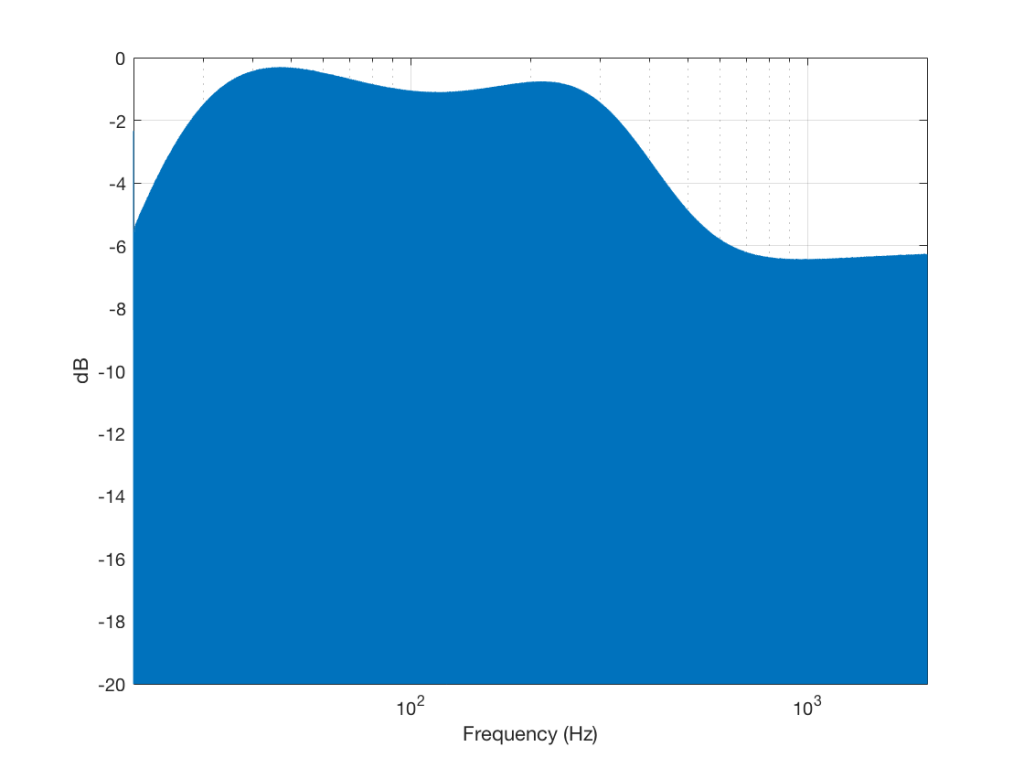Fig 6. the equivalent of Figure 1, but with bass boost set to ON on the mobile device. Again, this can be read as a frequency response plot – but notice that the vertical scale is now 20 dB.

Zooming in on the same 40 Hz region, we see the following: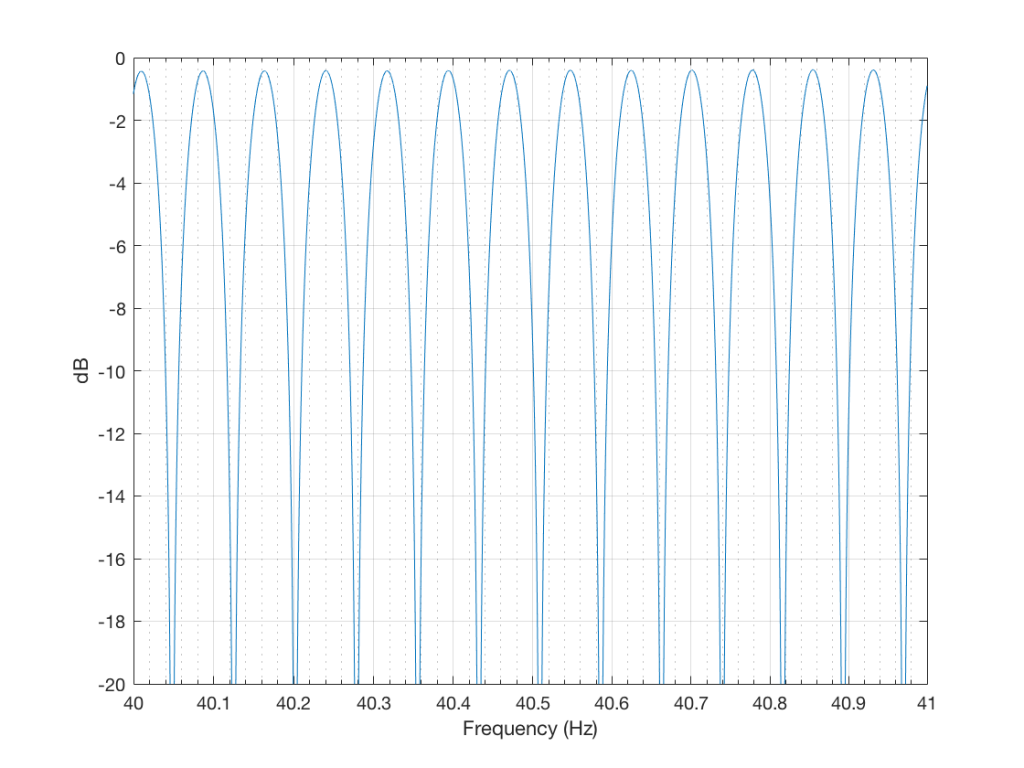Fig 7. An equivalent to Figure 2 – but with the bass boost set to ON on the mobile device. Again, it’s nice to see that there is no significant clipping…

So, as can be seen in those plots, the clipping problem that used to be quite obvious in some mobile devices has been corrected on this newer device due to a difference in the way the signal processing is implemented in the software.

However, as can be seen in Figure 6, this solution to the problem was to drop the midrange and treble by about 6 dB. (It’s also interesting that we lose almost 6  dB at 20 Hz when we think that we are boosting the “bass” – but that’s another discussion…) This might be considered to be a smart solution, since the listener can just turn up the level to compensate for the loss, if they wish. However, it does mean that, on this particular device, with this particular software version, if you do turn on the “bass boost” function, you’ll lose about 6 dB from your maximum level (which is equivalent to 4 times the sound power) in the midrange and high frequency regions (say, from about 600 Hz and up, give or take…). So, distortion has been traded for a lower maximum output level in the comparison between these two devices and software versions, two years apart.

So, generally speaking, it seems that I have fallen victim to exactly the problem that I often warn people to avoid. I believed the same thing on Wednesday that I believed on Monday… Then again, I know for certain that there are many people who are still walking around with the same distorting software / device that I complained in the “old days” (those first set of measurements are only 2 years old…). And, if you’re concerned about maximum output levels, the “new” solution might also not be optimal for your preferences.

So, it seems that “it depends” is still the safest answer…

# 997 Hz?

In my previous posting, I mentioned that I was using a tone at or around 997 Hz to test my signal. In truth, only one of the plots I showed there actually used 997 Hz – but that doesn’t really matter.

The question that I’ll talk about in this posting is “why did I prefer to use 997 Hz instead of 1 kHz as my target frequency?” (I didn’t just randomly choose 997 Hz – it’s a common number that’s often used by people in the audio industry.)

The answer to that question has to do with some considerations on how digital audio equipment and software is tested.

Let’s start by talking a little about how a signal gets a PCM (Pulse-Code Modulation) representation in the digital domain. Note that this is the VERY basic explanation – I’m leaving out a lot of steps here…

We’ll start with a signal like the portion of a sine wave shown in Figure 1.

This signal is continuous – meaning that we can zoom in infinitely and still get a smooth curve – both in terms of time, and amplitude.

We then take that signal and measure its amplitude every time a clock ticks – and regular intervals. This is represented by the red dots in Figure 2. (I just left out a whole lot of information about anti-aliasing filters, but it doesn’t matter for the purposes of this discussion…)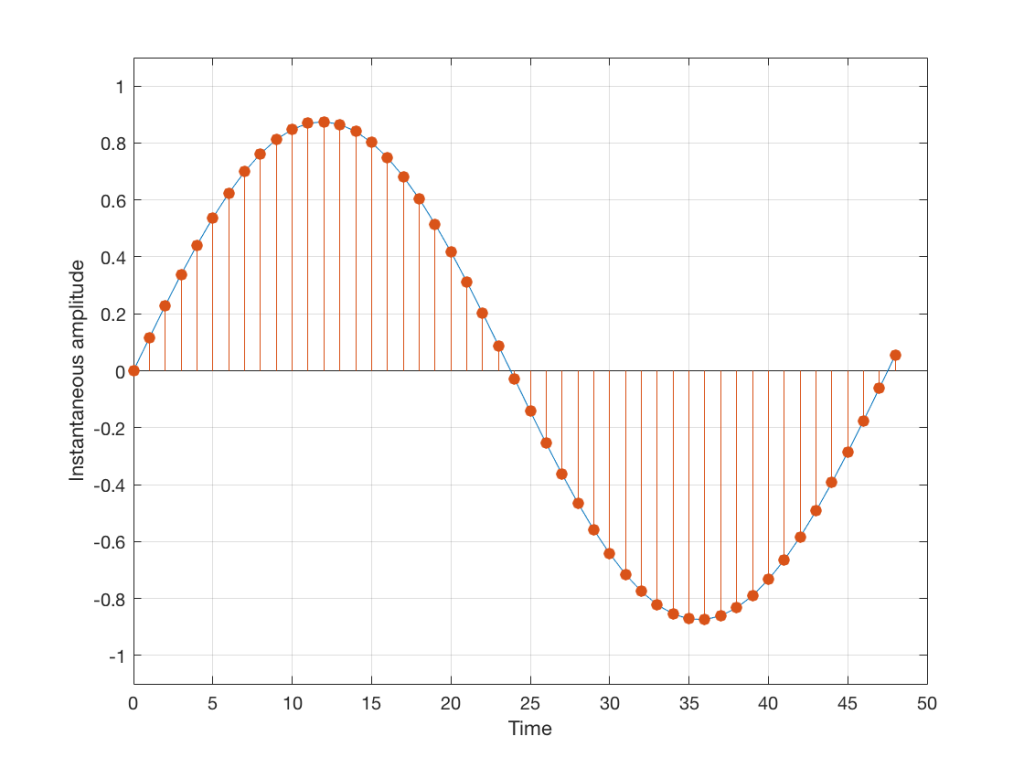Fig 2: An audio signal (blue) that has been sampled at discrete time intervals, but still has infinite resolution in its amplitude measurement (shown in red).

So, in Figure 2 we have a representation of a sinusoidal wave that has been “sampled” – a word that means “measured at regular time intervals. We are grabbing a “sample” or a “measurement” of the amplitude of the signal.

The problem is that the “ruler” we use to measure those values doesn’t have infinite resolution – just like the ruler that you would use to measure the length of something. If your ruler has lines only as fine as millimetres or 1/16th of an inch, then you cannot measure something accurately to the micrometer or to 1/64th of an inch. So, you “round off” your measurement to the nearest value on the ruler.

We do the same with audio – we have a finite number of values that we can store or transmit to represent the instantaneous amplitude of the signal, so we have to round off or “quantise” the values to the nearest value that we have. The result looks something like Figure 3: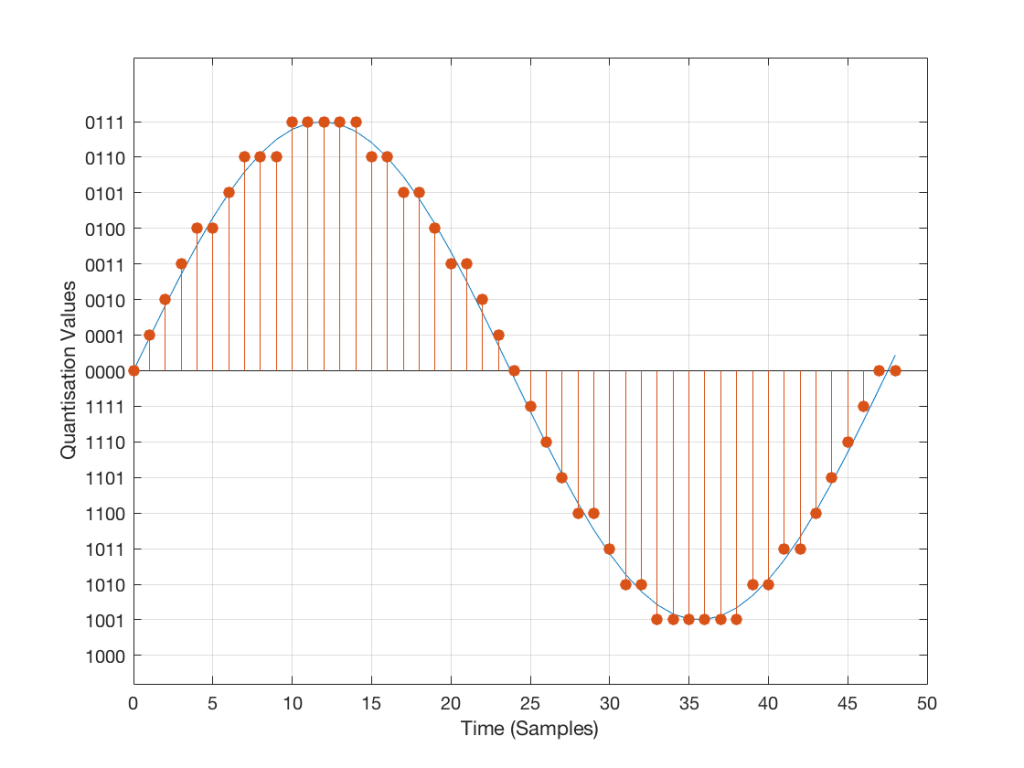Fig 3: An audio signal (blue) that has been sampled at discrete time intervals and “quantised” or “rounded off” to the nearest available amplitude value (red).

I’ve shown the quantisation values on the left (the Y-axis) as binary values. As you can see there, we have a 4-bit signal which gives us a total of 2^4 = 16 possible quantisation values for storing the signal’s amplitude at each sample.

If you’re really paying attention, you’ll notice that there are one fewer positive values than negative values, since one of the positive values is taken to represent the “0” line. This is why, when I made my original signal, I didn’t scale  it all the way up to ±1 – just to keep things smooth in the explanations. If you aren’t paying that much attention, and you didn’t notice this – then please have a look, since it will come up again later…

Normally, of course, we store audio signals with a LOT more bits than this – a CD uses 16-bit resolution, which gives us a total of 65536 possible quantisation levels (2^16). Other systems use a different number of bits – either fewer or more, depending.

At this point, it should be pretty clear that you have a finite number of samples (or measurements) per second (typically 44100 samples per second (or 44.1 kHz), if it’s a CD, although 48000 samples per second (48 kHz) is also a pretty common number – other systems use other values for this.)

So, if we look at a CD, we have 44100 samples per second, and  65536 possible quantisation values to choose from for each sample (because it’s a 44.1 kHz, 16-bit system). Notice that we have more quantisation values than samples per second…

Now, let’s say that we want to test a piece of digital audio gear, and one of the tests that we wanted to perform was to ensure that all possible quantisation values are working properly (whatever that means). Let’s also say that the gear has only 4 bits of resolution and is running at a sampling rate o 48 kHz, to start. One way to test any audio gear is to feed in a sine tone and to see what comes out. So, we’ll do that, using a 1 kHz sine tone. The result looks like Figure 4, below.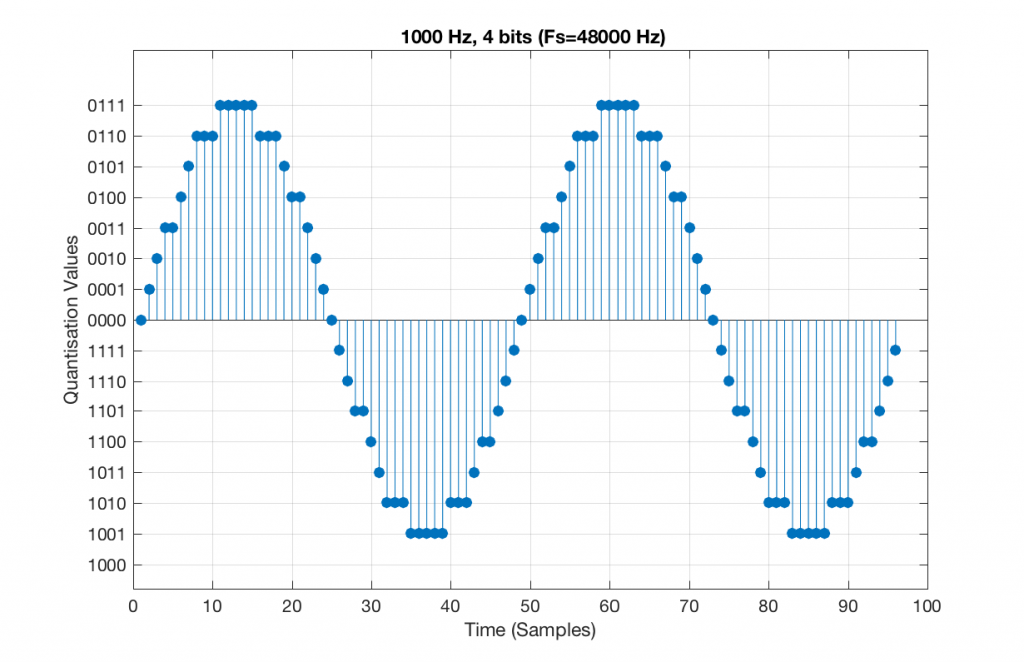Fig 5. A 1 kHz sine tone, represented in a PCM system with 4 bits of resolution and a sampling rate of 48 kHz.

There are two things to notice about that signal in Figure 5:

• The first is that all possible quantisation values are used at least once – except for the very bottom one – but that last one is my fault, caused by the scaling of the sine wave, and the fact that it is symmetrical.
• The second is that the wave is perfectly periodic – meaning that it repeats itself over and over and over… There are two cycles of the waveform shown in the plot, and if you count the dots, you’ll see that the two are identical. This second point is the one that will be important to understand as we go further. The reason this exact repetition happens is because the frequency of the sine tone (1000 Hz) is an integer divisor of the sampling rate (48000 Hz). In other words, 48000 / 1000 = 48 – not a weird number like 48.3.

Let’s take that same signal (1 kHz in a 4-bit, 48 kHz PCM system) and we’ll count the number of times each sample value occurs after 1 second (or in a time of 48000 samples). We can then plot these values as is shown in Figure 6, which is a kind of plot called a “histogram”.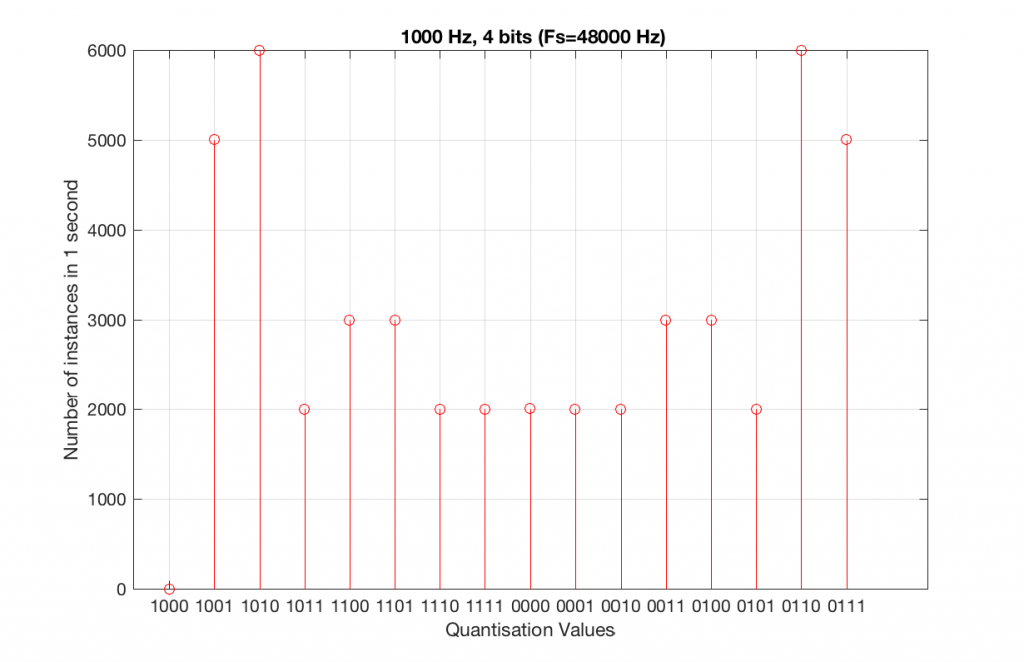Fig 6. A histogram of the number of times each quantisation value is used in 1 second of a 1 kHz sine tone in a 4-bit, 48 kHz PCM system.

As can be seen in Figure 6, the bottom quantisation value (1000) is never used – but apart from that one, all others are.

Let’s do the same thing, but with a 4-bit, 44.1 kHz system instead. The results of this are shown below in Figure 7 and 8.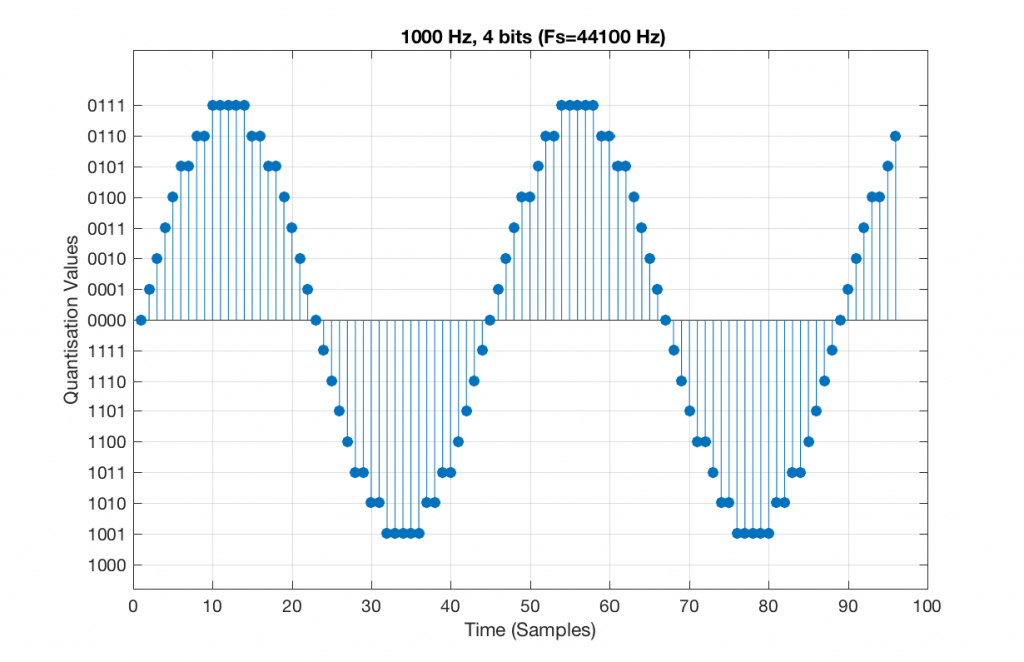Fig 7. A 1 kHz sine tone, represented in a 4-bit, 44.1 kHz system. Notice that the second instance of the waveform is not identical to the first. This is because 44100 / 1000 = 44.1 – not an integer value.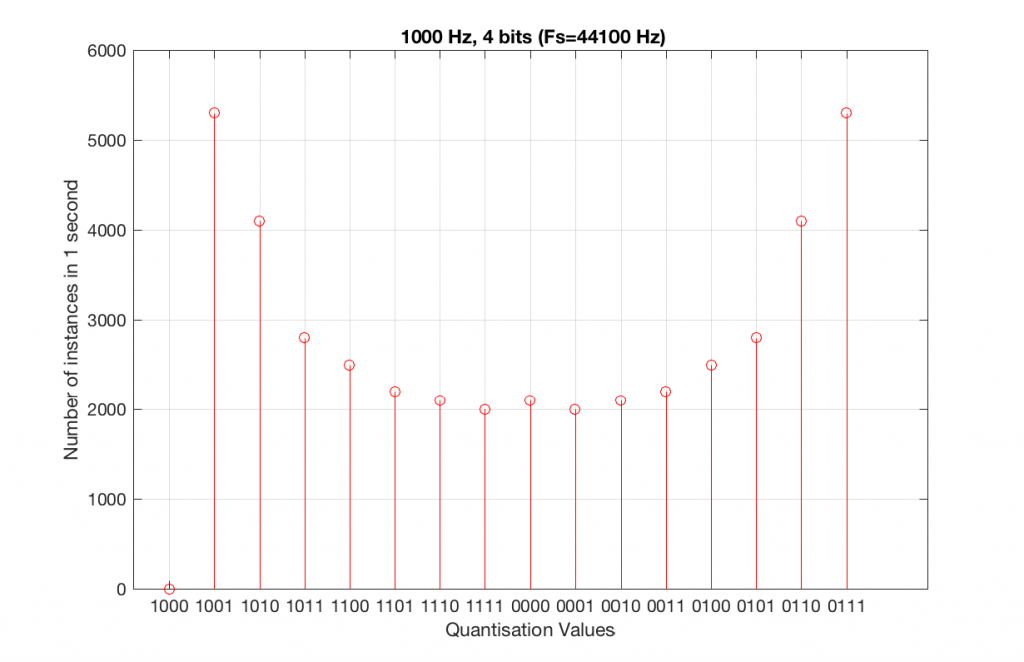Fig 8. A histogram of the quantisation values of 1 second of a 1 kHz sine tone in a 4-bit, 44.1 kHz PCM system.

Compare Figures 6 and 8. Notice that Figure 8 appears to be a “smoother” shape. This is due to the fact that the instances of the waveform are not identical copies of each other. As can be seen in Figure 7, the waveform is slightly different. Of course, after a full second, then the whole cycle repeats itself, since there are 1000 cycles per second in the signal, and 44100 samples per second. If the signal were 1000.1 Hz, then it would take 10 seconds for the repetition to start.

Let’s increase the number of bits and see what happens. We’ll take it up to 6 bits.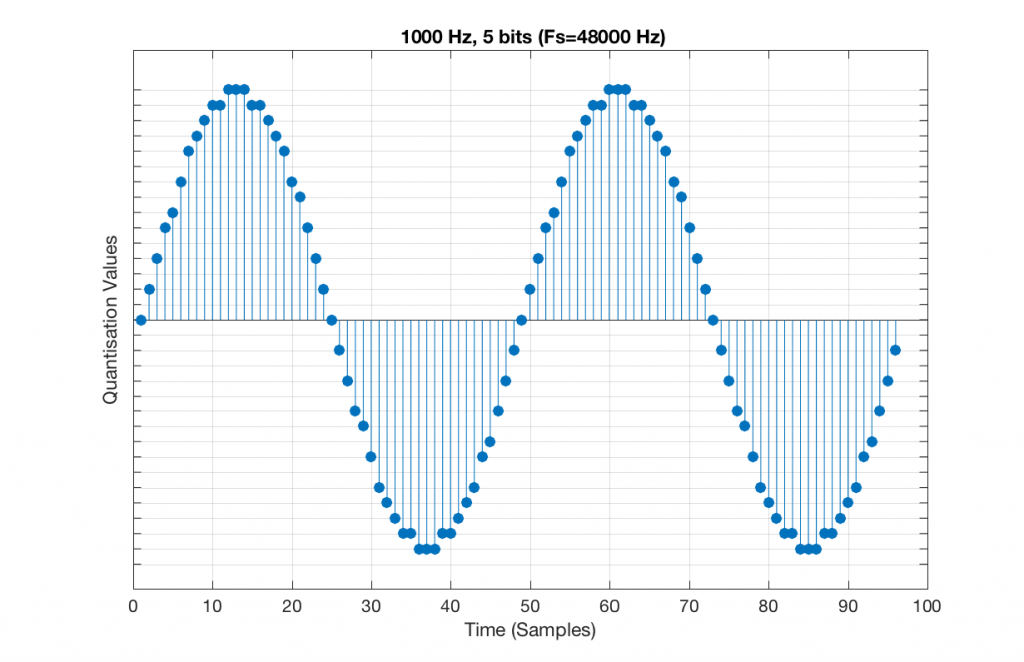Fig 9. A 1 kHz sine tone, represented in a PCM system with 5 bits of resolution and a sampling rate of 48 kHz.

Figure 9 shows a 1 kHz sine tone in a 5-bit, 48 kHz system. Again, since 48000/1000 = 48, the two cycles are identical to each other. However, something new has happened here. If you look carefully at the positive side of the sine wave, you may notice that there are 5 quantisation values that are never used. On the negative side, there are 3 unused values, as well as the very bottom one.

So, because we are in a 5-bit system, we have 2^5 = 32 possible quantisation values, but, because we are using a 1 kHz sine tone, 9 of those possible values are never used. As a result, our histogram looks like Figure 10, below.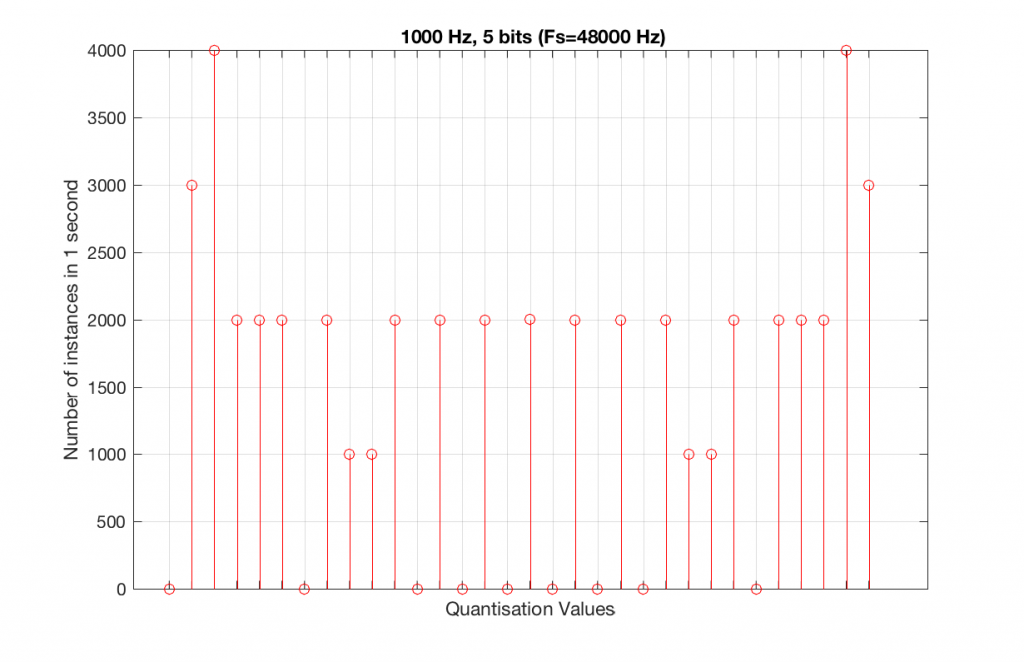Fig 10. A histogram of the quantisation values of 1 second of a 1 kHz sine tone in a 5-bit, 48 kHz PCM system. Notice that 8 of the possible 32 values are not used (plus one more at the bottom).

Let’s now compare that to a 5-bit, 44.1 kHz system.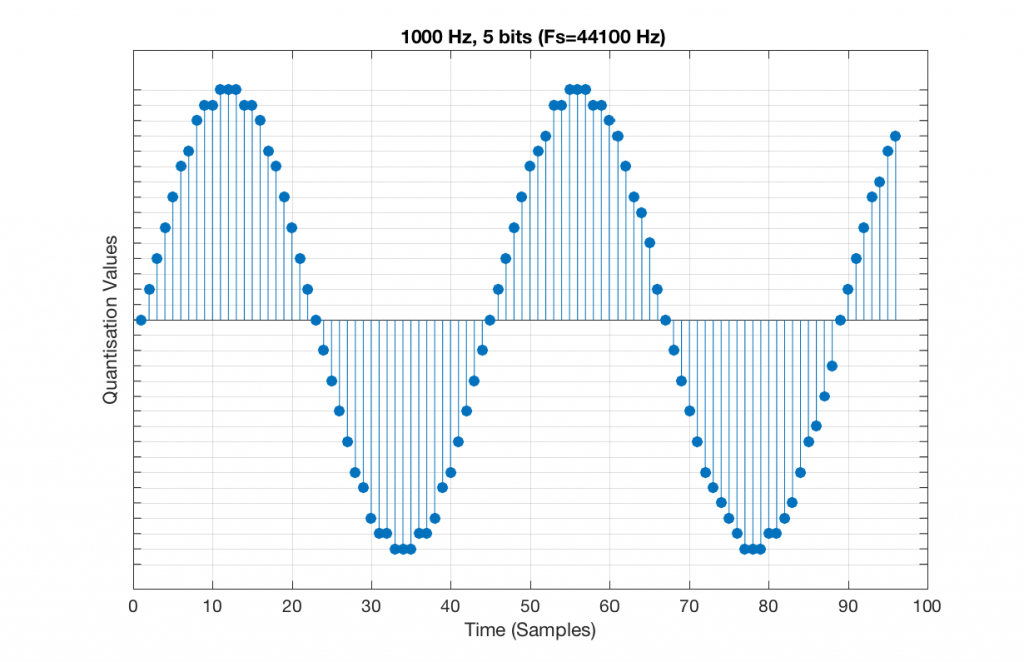Fig 11. A 1 kHz sine tone, represented in a PCM system with 5 bits of resolution and a sampling rate of 44.1 kHz.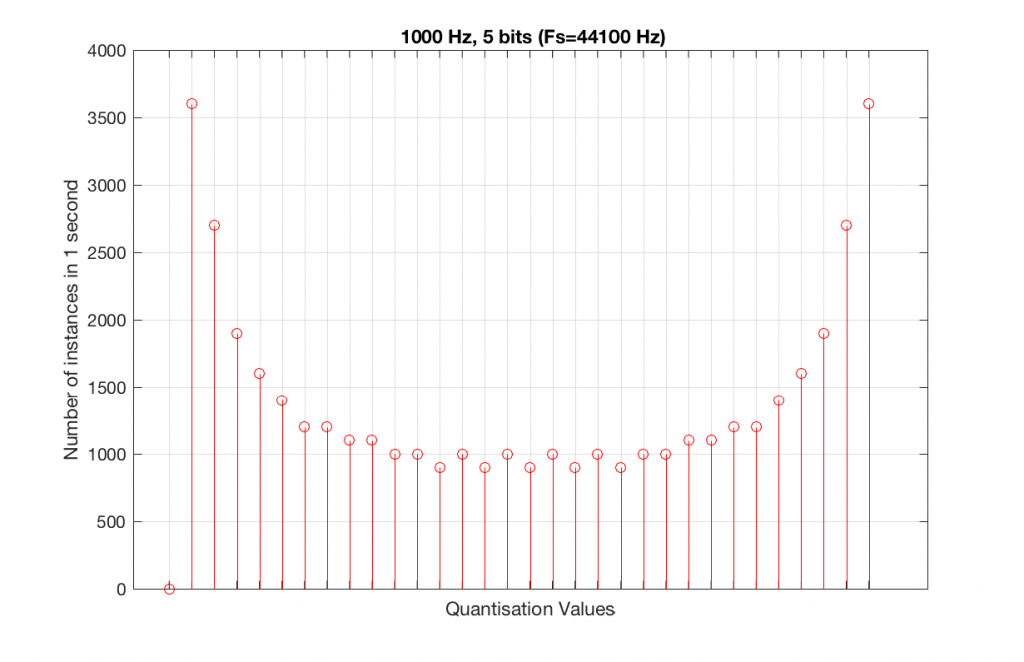Fig 12. A histogram of the quantisation values of 1 second of a 1 kHz sine tone in a 5-bit, 48 kHz PCM system. Notice that all of the possible 32 values are used (except for the bottom one…).

We can see that there is a basic problem here. The behaviour of the system may be different due only to the relationship between the sampling rate and the frequency of the signal.

The question is “what do we do about this?” We can see from Figures 10 and 12 that, when the signal’s frequency is not a nice round divisor of the sampling rate, we stand a better chance of testing the system more completely. So, instead of using a “nice” frequency like 1000 Hz, let’s use something close, but different enough to make things “misbehave” a little. One possible solution is to use 997 Hz, as we can see below: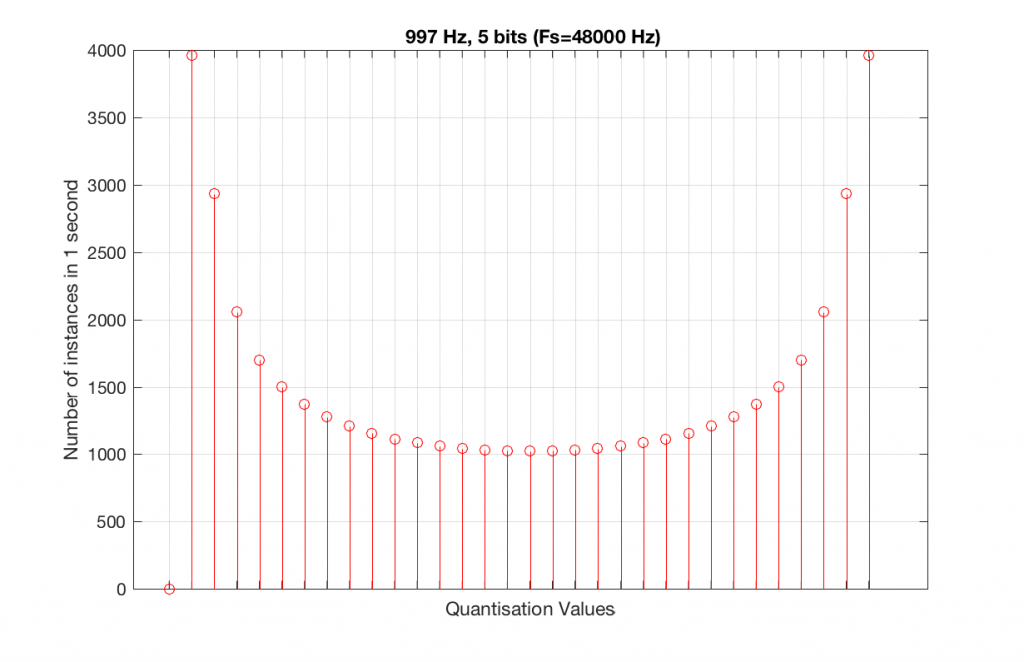Fig 13. A histogram of the quantisation values of 1 second of a 997 Hz sine tone in a 5-bit, 48 kHz PCM system. Notice that all of the possible 32 values are used (except for the bottom one…).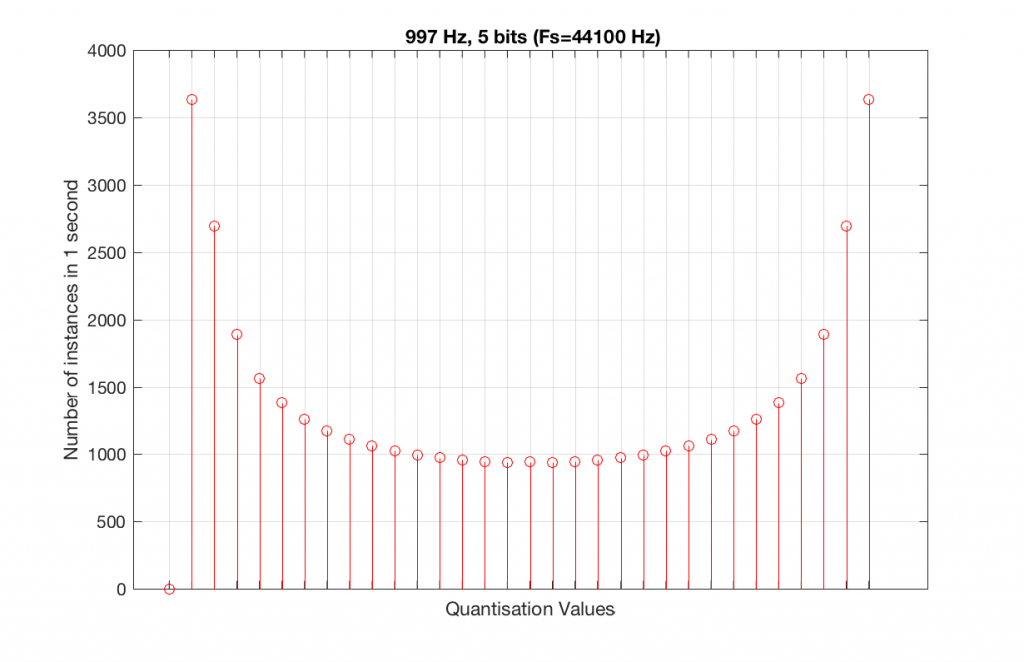Fig 14. A histogram of the quantisation values of 1 second of a 997 Hz sine tone in a 5-bit, 48 kHz PCM system. Notice that all of the possible 32 values are used (except for the bottom one…).

As can be seen in the histograms in Figure 13 and 14, changing the signal to 997 Hz from 1000 Hz results in us using all of the quantisation values in both sampling rates. So, we do a more thorough test, and stand a better chance of not missing anything…

At this point, you might say, “yes, but normally we used far more than 5 or 6 bits – this won’t happen in a system with more bits…” Nice try, but actually, things get worse, as you can see in Figures 15 and 16, below.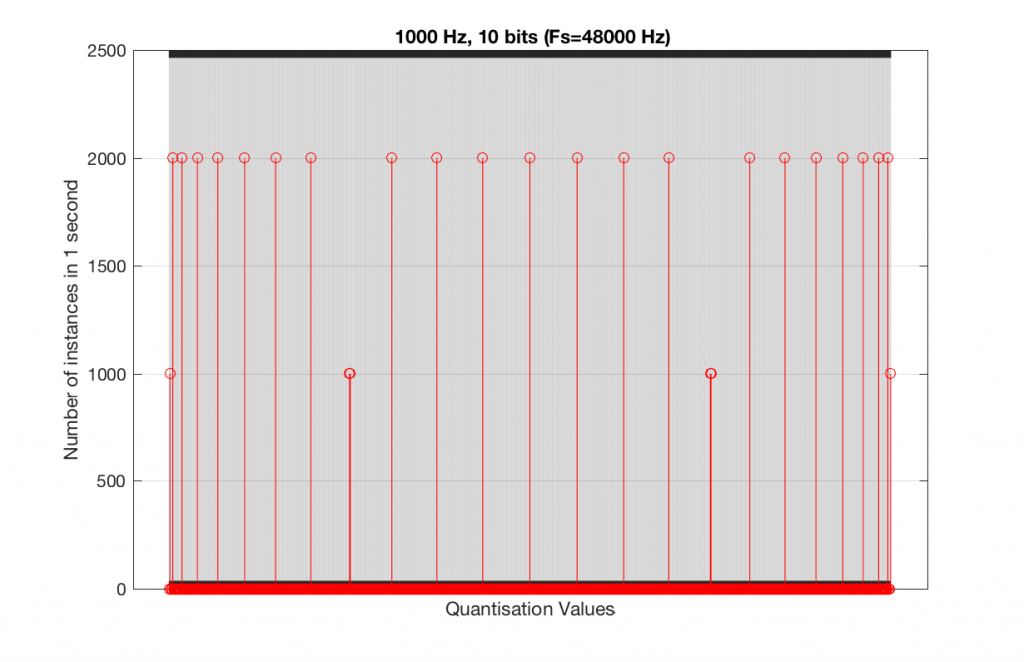Fig 15. A histogram of the quantisation values of 1 second of a 1 kHz sine tone in a 10-bit, 48 kHz PCM system.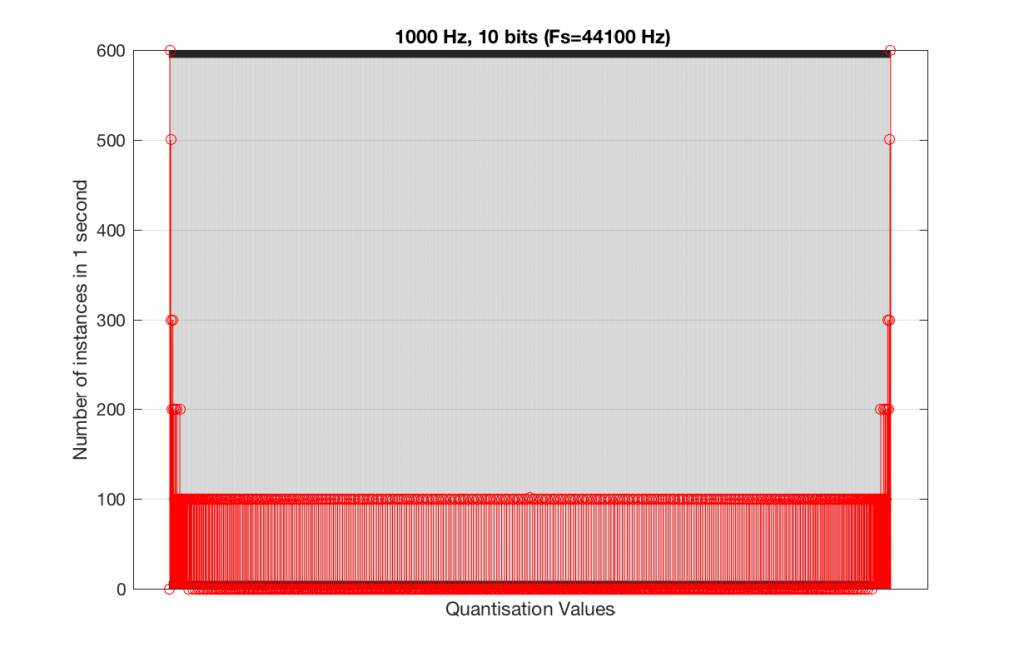Fig 16. A histogram of the quantisation values of 1 second of a 1 kHz sine tone in a 10-bit, 44.1 kHz PCM system.

As you can see in Figures 15 and 16, lots of quantisation values are unused in both sampling rates with a 1 kHz signal. By comparison, if we used a 997 Hz tone, the results would be very different, as is shown in Figures 17 and 18.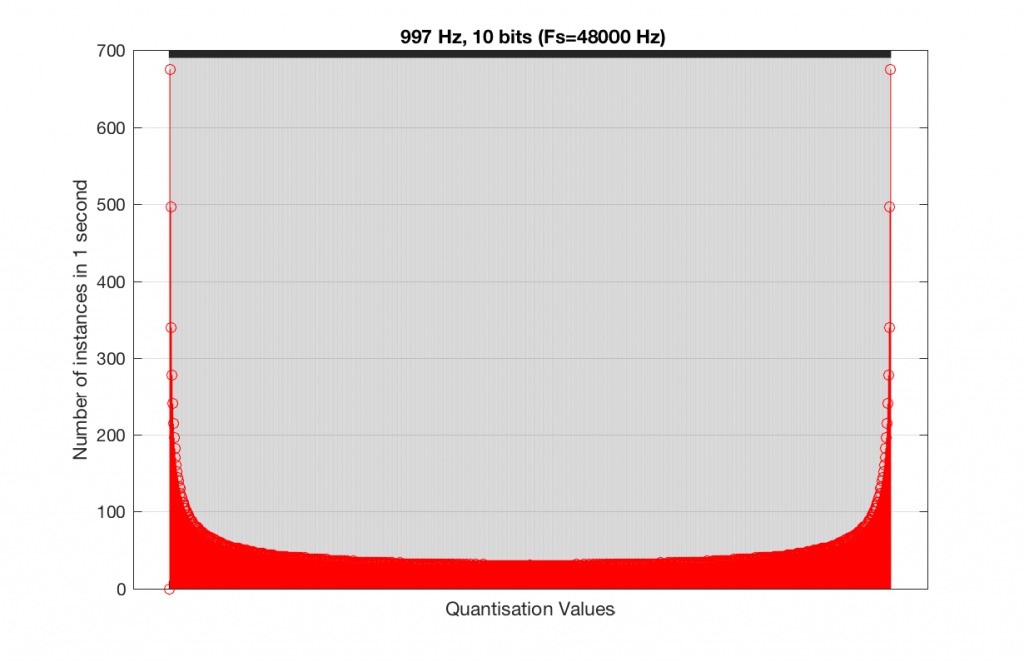Fig 15. A histogram of the quantisation values of 1 second of a 997 Hz sine tone in a 10-bit, 48 kHz PCM system.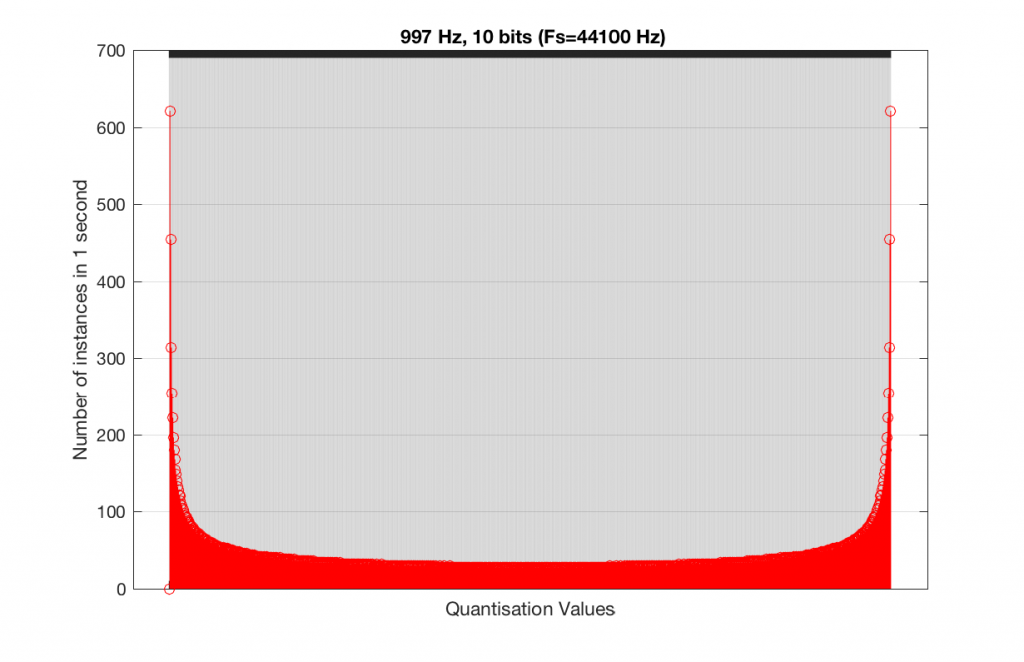Fig 16. A histogram of the quantisation values of 1 second of a 997 Hz sine tone in a 10-bit, 44.1 kHz PCM system.

In fact, as we get more and more bits of resolution, the worse the problem gets, since we have an increasing number of available of quantisation values (increasing by a factor of 2 every time we add another bit), but the number of values that we use does not increase.

This is because, at some time, we start repeating the cycle. If the sampling rate divided by the signal frequency is an integer value (like a 1 kHz tone in a 48 kHz system), then we don’t use any new quantisation values after the first cycle of the tone (or 1 ms, in this case). If the sampling rate divided by the signal frequency is not an integer value (like a 997 Hz tone in a 48 kHz system) then we don’t start repeating ourselves until 1 second has passed.

However, think back to a comment that I made up at the top – if signal does start repeating itself after 1 second (in other words, if the frequency is an integer value), and if the number of samples per second is smaller than the number of quantisation values, then we will start repeating ourselves after 1 second, and we will only test the number of quantisation values that is equal to the sampling rate.

For example, if you have a 16-bit system, then you have 65536 possible quantisation values. If the sampling rate is 48000 Hz then we could only test a maximum of 48000 possible quantisation values out of the 65536 possible ones in one second, regardless of the frequency that we choose. Typically, however, we test fewer than this, because of the repetition of some values (e.g. the maximum value, if you have a periodic signal with a frequency greater than 1 Hz).

If we do this for the two frequencies we’ve been looking at – 1 kHz and 997 Hz, for two sampling rates, 44.1 kHz and 48 kHz, at different bit depths, the results look like the following figures.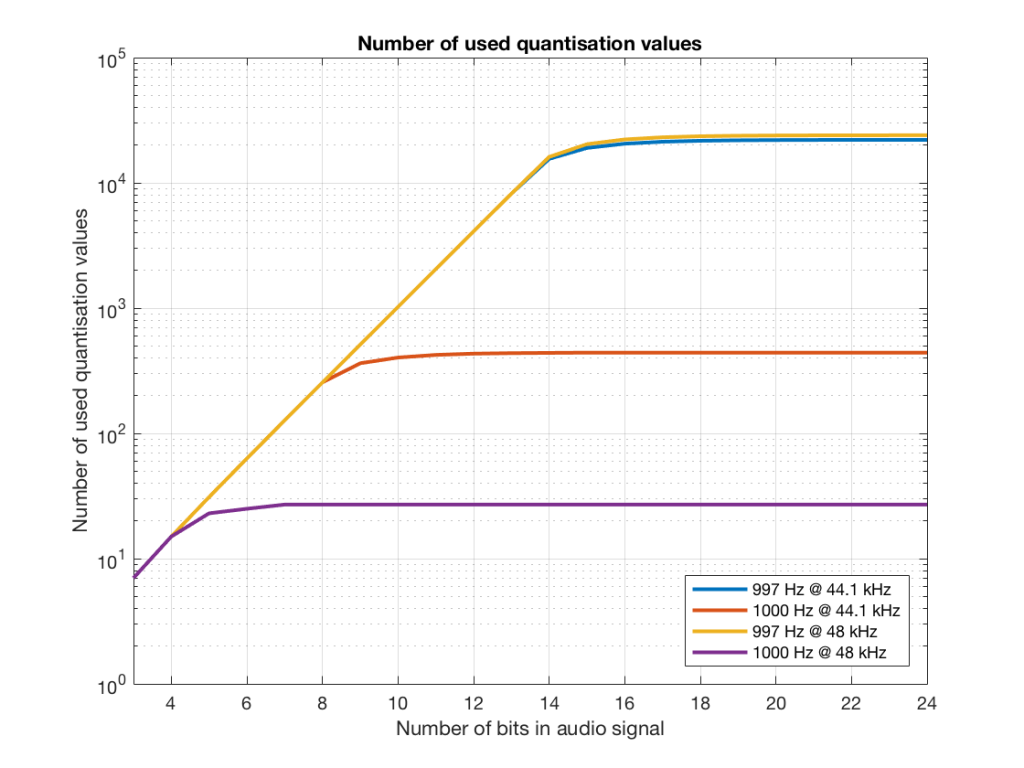Fig 17. The number of quantisation values used for 997 and 1 kHz tones in PCM systems with sampling rates of 44.1 or 48 kHz, for varying bit depths.

Notice in Figure 17 that the total number of quantisation values that are used when you have a 1 kHz tone in a 48 kHz system does not increase once you hit a word length of 7 bits. That does not mean that the signal’s representation does not improve – it does, since the quantisation values that you are using have a better resolution – so you’re rounding off less, so the error is smaller.

Notice as well that the 997 Hz tone not only results in us using far more quantisation values (topping out at the sampling rates) than the 1000 Hz tone, but that they are more similar in the two sampling rates.

If we plot the number of unused samples instead, it looks like Figure 18.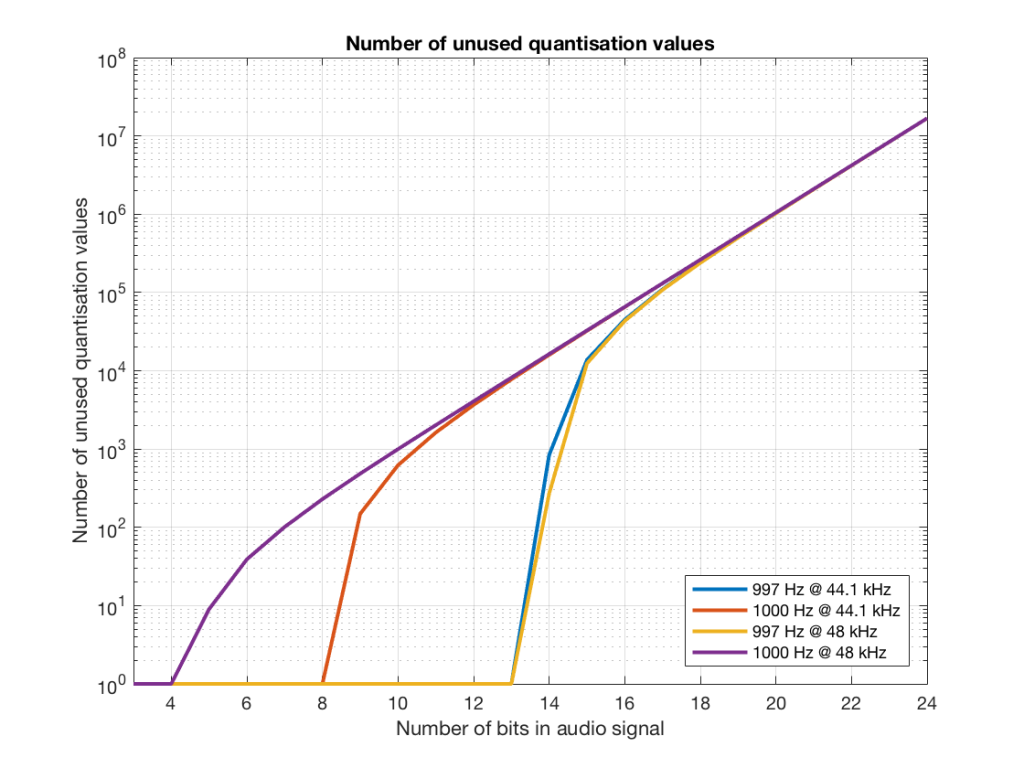Fig 18. The number of quantisation values that are not used for 997 and 1 kHz tones in PCM systems with sampling rates of 44.1 or 48 kHz, for varying bit depths.

Figure 18 is a little misleading, since as the bit depth increases, the total possible number of quantisation values also increases, however, since the two frequencies that we are analysing are integer values, the maximum number cannot go past the sampling rate. So, in an extreme case (if you choose your frequency or signal carefully), only 48000 values out of a possible 16777216 values are used in a 24-bit system per second in a system with a sampling rate of 48 kHz.

Figure 19 shows the same information as Figure 18, except that I’ve displayed the values in percent.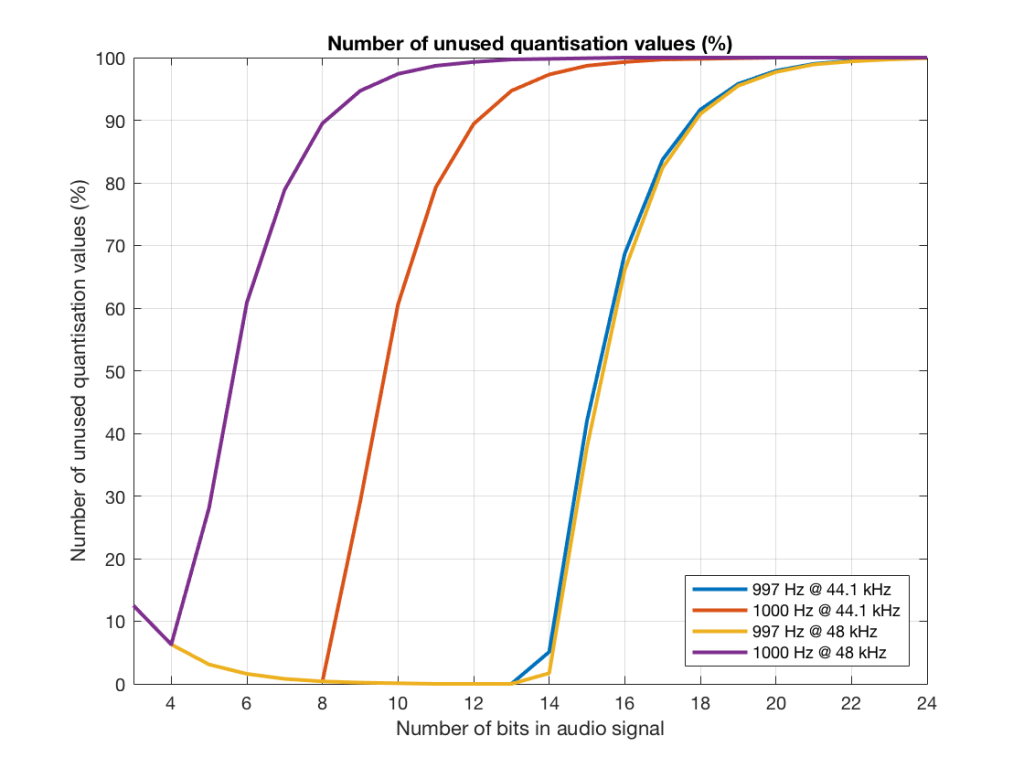Fig 19. The percentage of quantisation values that are not used for 997 and 1 kHz tones in PCM systems with sampling rates of 44.1 or 48 kHz, for varying bit depths.

So, as you can see there, in a 16-bit system, even if you use a 997 Hz tone, about 70% of the total possible quantisation values are used.

Caveat

Of course, the signals that I used here were generated digitally, and did not include dither. If I had included proper dithering, then more of the quantisation values would have been used. However, the point of this posting was not to talk about correct ways of creating PCM signals – it was an attempt to explain why we use 997 Hz instead of 1 kHz when we test digital audio systems.

Sometimes, someone will use a plot to show the relative levels of different frequency bands in a signal. Even I have done this from time to time…. However, it’s important to have the skills to be able to read these plots with a little-more-knowledge-than-normal in order to not be distracted into thinking something that isn’t true.

One way to calculate the relative levels of frequency bands of a signal (whether it’s a measurement of a loudspeaker, a black box, or your favourite track on your favourite CD) is to so something called a “Fourier Transform”. This is a set of calculations that can be used to show how much energy there is in a signal, by frequency.

Typically, we do a Discrete Fourier Transform or “DFT” – although most people call it a Fast Fourier Transform or “FFT”. We will not discuss the difference between these things in this posting. I’ll just use the term “FFT” here, in order to be like everyone else…

(If you’d like to know how to do your own FFT’s by hand, this is one place to start learning…)

In order to give me something to analyse, I made a signal comprised of a sine tone with a frequency around 997 Hz. (I’ll explain at the end why it’s not exactly 997 Hz. I’ll also explain in another posting why I chose 997 Hz instead of a good-old-fashioned 1 kHz.)

I set that sine tone to have a level of -1 dB FS.

Then, I made some white noise and set its level to be exactly 80 dB below the level of the sine tone. In order to calculate this, I found the total RMS value of the noise signal, and used this to create a gain that makes it a level of -81 dB FS. (Just for the sake of being as pedantic as possible, the white noise that I created was the result of a “rand” function in Matlab, which, as you can see in this posting, has a rectangular probability density function.)

Therefore, I have an input that has a signal-to-noise ratio of 80 dB. (Note that this measurement does not use any band-limiting on the white noise… Typically a SNR measurement would apply some low pass filter to the noise.)

To keep things looking pretty on my graphs, I set the sampling rate to 65536 Hz (2^16).

Then, I pretended that this signal was coming in from some unknown device, and I do an FFT on it to find out the relative balance between the signal (the sine tone) and the noise (which I already “secretly” know is 80 dB lower)

If I do an FFT of 256 points on the signal (and therefore, I’m only looking at the first 256 samples of the signal – this is an important point that we’ll come back to later…), the result looks like Figure 1.

Note that the sine tone is a little higher than 997 Hz – but this is not really important. (the explanation is at the end!).

There are some things to notice here:

The first is that the plot does not extend lower than a frequency of 256 Hz. This is because the resolution of a 256-point FFT is 256 Hz – so, there is a “point” on the plot every 256 Hz – typically called a “bin” – since it contains information about the level of a collection of frequencies around its centre frequency. (If you’re new to FFT’s, don’t jump to the conclusion that the frequency resolution is equal to the length of the FFT. This is incorrect. The frequency resolution is equal to the sampling rate divided by the FFT length – 65536 Hz / 256 bins  = 256 Hz.) Limiting the length of the FFT limits its resolution, which has an obvious impact when we plot the results on a logarithmic frequency scale.

The second is that, although the SNR of the signal is 80 dB, on the magnitude response, it appears that the noise is generally lower than -100 dB. This is not that difficult to believe, since the noise is spread over a wide frequency range – so, although any one frequency may, indeed, be more than 100 dB below the signal – the sum of the energy in all of those frequency bands totals more than any of the individual contributions. (In the same way that 1000 people can shout louder than 1 person – even if all 1000 people are, individually, shouting at the same level.)

One thing that is not obvious from the plot, but that we have to keep in mind is that this shows us the level of the different frequency ranges over the entire length of the signal (all 256 samples of it). However, the noise that I created that is part of that signal is exactly that – noise. Since it is noise, there is no guarantee that all frequencies are represented at the same level at any one time – in fact, they’re not. “White noise” has the characteristic of having equal probability of having the same level at all frequencies. But if 1000 people have equal probability of winning a lottery, that doesn’t mean than 1000 of them will win. In order to ensure that you actually get the same level at all frequencies, you would have to listen to white noise forever – and I’m not willing to wait that long…

Figure 2 shows the same analysis done on the same signal, but with a 512-bin FFT instead. There, you can see that the the resolution of the plot is better – we have a bin or point every 128 Hz (remember 65536 Hz / 512 bins = 128 Hz). Also, the sine tone has the same level (-1 dB FS) but the noise, which we know is 80 dB lower, appears to be even lower than it does in Figure 1… Strange…

Let’s do some more FFT’s with more and more bins to see what happens…

So, by going from a 256-bin FFT to a 65536-bin FFT, we appear to have dropped the noise floor by more than 20 dB.

Weird? No. Why?

Remember that every time we double the length of the FFT, we double the number of frequency bins in its output. So, that plot in Figure 9 has more individual frequencies contributing to add together to the same noise signal, 80 dB lower than the sine tone. (If you asked 1000 people to shout as loudly as 10 people, each individual in the larger group would have to be quieter to produce the same total output.)

The “punch line” here is that we cannot make a direct conclusion about the overall Signal-to-Noise ratio of the signal by looking at any of the plots above. Of course we can say that the “signal” (the sine tone) is obviously louder than the noise – by a lot. But we can’t be much more detailed than that.

So, if someone jumps between a SNR number and a spectral plot like the ones above, in an effort to convince you of something, be very careful about being led down a garden path.

Some extra information:

We also have to remember that, although the signal that I used to make these graphs was initially the same, the actual signal that was used by each of the FFT’s was different. This is because, by default, the length of the signal used by an FFT calculation is the same as the number off bins in the FFT. So, for example, a 256-bin (or 256-point) FFT only uses 256 samples as its input. A 32768-point FFT uses 32768 samples (the first 256 of which were the ones used by the 256-point FFT).  So, for example, if you load a recording of Britney Spears singing “Toxic” into Matlab, and you type the command FFT(toxic, 256) – you’ll get a 256-bin FFT of the first 5 .8 milliseconds (256 samples) of the recording  – not a representation of the spectral content of the entire song.

Initially, I started out by saying that I would use a 997 Hz sine tone. This might look a little weird because it’s not a nice number like 1000 Hz. There’s a good reason for this, and I’ll write a posting about it some other day.

Then, I said that it’s not really 997 Hz – I moved it a little. This is because I wanted the frequency of my sine tone to land exactly on one of the bins of my FFT. So for example, in the case of the 256-bin FFT, I had a frequency resolution of 256 Hz – so my bins are at the following:

256 Hz
512 Hz
768 Hz
1024 Hz

1024 Hz is the closest value to 997 Hz that occurs in the sequence so I used that instead. If I had kept the sine tone fixed at 997 Hz, the plots would not have looked as pretty, because the information about its level would have “leaked” or “been spread out” into the adjacent bins. So, instead of a nice clean spike, we would have seen a big, round bump.